# Brainhack 2021 - CV and SC Competition## DSTA Brainhack 2021: Object Detection and Speech Classification

Having participated in Brainhack 2020, my team and I decided to come back for the online sequel - COVID edition! This year, we were required to develop robust Object Detection models and Speech Classification to classify the word in a given audio clip. The preliminaries were held over a month, where the top teams were invited to a week-long final.

Fortunately, our team had built a sufficiently robust machine learning pipeline that allowed us to easily enter the finals with our 1st place public leaderboard scores. During the finals, datasets were released every two days with an increasing number of classes and variations in the train/test set. Here’s how we managed to clinch first place: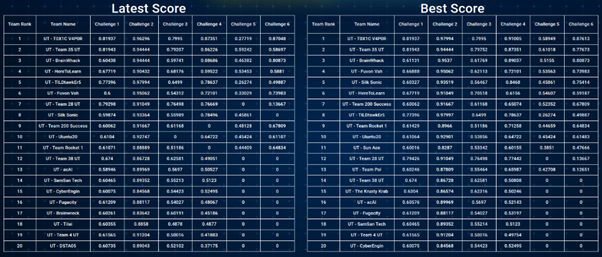### Object Detection

For the object detection challenge, our team decided to use the Detectron2 framework by Facebook Research. Many bleeding-edge models such as UniverseNet and VariFocalNet were available and easy to deploy using this technology, allowing us to start from pre-trained benchmarks. This allowed us to save a lot of time training with the limited hardware we had available from Google Colab, for this time-constrained competition.

Before starting each stage of the challenge, we had to merge the new datasets into the existing ones. Fortunately, everything was in the COCO format, so it was easy to do so with a simple python script, which allowed me to iteratively append new data with sequential IDs.

For the actual data pipeline, we used Detectron2’s in-built Albumentations library to add augmentations to the train set, including ShiftScaleRotate, RandomBrightnessContrast, JpegCompression, and normalised the data to fit our model input layer. Since we were running the training using Detectron2’s command-line interface with a python configuration file, it was relatively straightforward to iterate and determine which set of configurations gave us the best result. Additionally, it made it much simpler to deploy additional training instances on platforms like Google Colab and AWS EC2.

After the augmentations, the model was trained on the data (with a separate set for validation) for 50 epochs, or until the test accuracy started to plateau, whichever was earlier to avoid overfitting.

For manual tuning, we decided to take a peek at the output of our model, with the bounding boxes overlaid on the image. We realised that there were some edge cases that the model consistently missed, such as a cluster of objects being under-classified as 2-3 instead of 5-6. I tried to add more data from the OpenImagesV6 dataset, but it didn’t improve the results significantly. In the end, I accepted those as edge cases that would be very hard to account for without significant changes to the model, which couldn’t be done in a short period of time. Otherwise, the model was actually doing pretty well!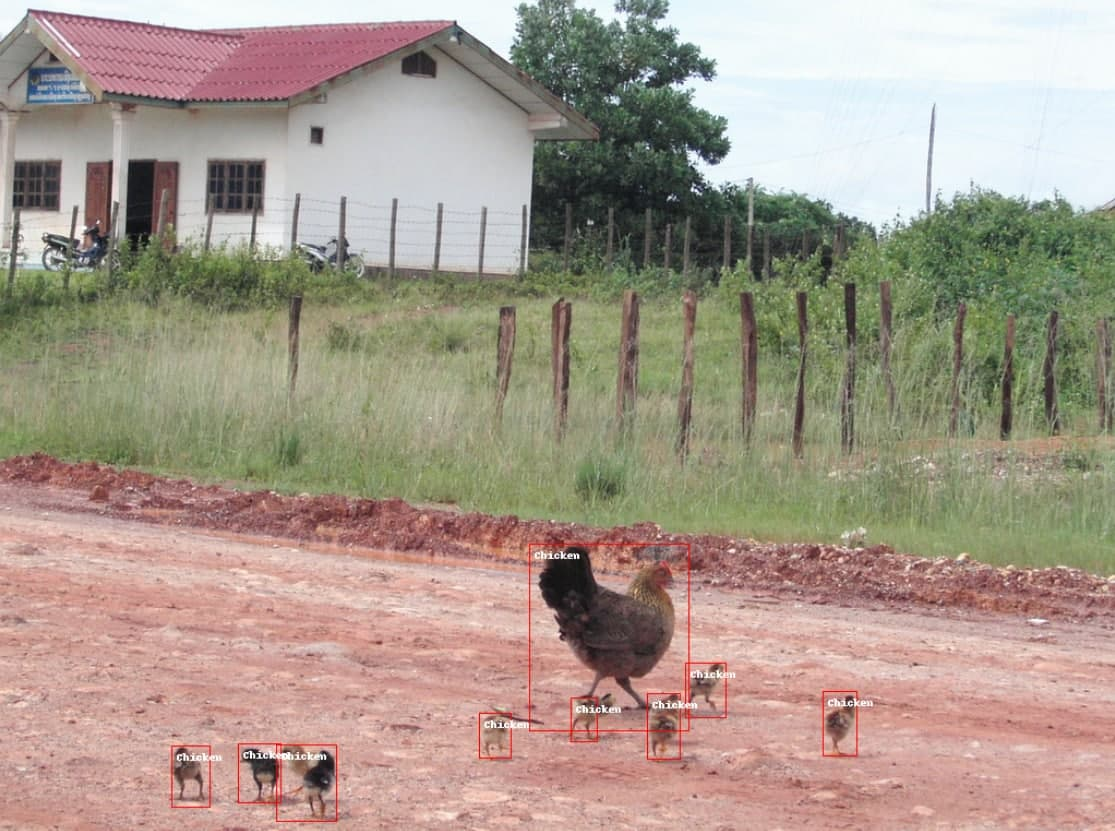One takeaway I had from this, was learning how to use AWS to quickly setup and deploy machine learning training. I made a short bash script to install the required dependencies and pull the data from my Google Drive using `gdown`, then another script to start training the model and automatically upload the trained model `.pth` files to Google Drive.

### Speech Classification

This challenge was a little more tricky. The first few challenges were pretty straightforward; run the audio through a Melspectrogram, then an Image Classification model, and you would get a model with accuracy >90%. A melspectrogram is a spectrogram obtained by taking the Fast Fourier Transform (FFT) of the audio clip, then adding a mel-filter to account for the sensitivity of human hearing to different frequencies. In this way, the model is able to focus on frequencies “important” to human hearing.However, in the last challenge, the clips started to sound “robotic”, much like the audio quality from a telephone line in the 90s. We immediately recognised it as a low-pass filter clipping off higher frequencies, similar to how old telephones didn’t have enough bandwidth to encompass higher tones. We added a band-pass filter to cut off frequencies out of the range of human hearing, as well as some of the higher frequencies. This helped us tremendously on the scoreboard, as we were able to pull ahead by several percentage points in test accuracy.

The image augmentations were broadly similar to that of object detection, once the audio was turned into a melspectrogram. The main difference was windowing the audio clip length, to generate a melspectrogram of correct input size to our model, rather than a simple resize.

### Conclusion

All in all, Brainhack 2021 was a great experience despite losing the hardware/robotics integration aspect due to COVID-19. My team and I gained a lot of experience in Machine Learning and deployment on web services such as AWS, and really look forward to tackling more, exciting challenges in the future!

# 60GHz Radar - GovTech Embedded Systems Internship## 60GHz Radar: Embedded Systems Sensors

In the summer of 2020, I had the opportunity to intern at GovTech Singapore Sensors and IoT. During that period I worked on a proof of concept with cutting-edge 60GHz mmWave radar sensors.

60GHz mmWave radar is a new type of sensor technology pioneered by Texas Instruments, by incorporating the entire radar front end in CMOS architecture, enabling an SoC design combining the RF front end, DSP for I/Q signal processing, and the CPU for general instructions.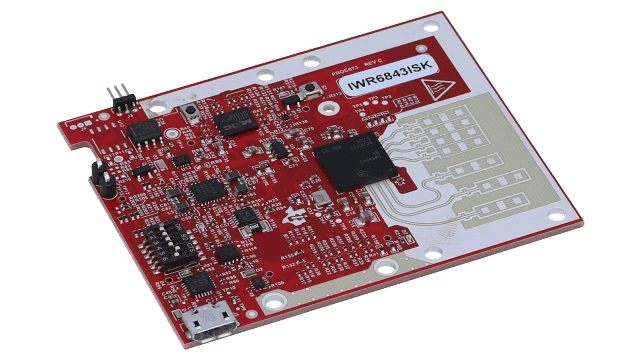In principle, what this radar does is send out a chirp, a specially modulated radio wave around its baseband frequency (60GHz), and “listen” for the reflected signal as it bounces off nearby objects. It’s similar to how you can shout into a large room and listen for the echo to determine how far parts of the room are from you. You can use the time delay between sent and receive pulses, as well as the beat frequency, sent through a Fast Fourier Transform (FFT) to determine the frequency of the received wave. This allows you to determine the Doppler and Signal-to-Noise ratio of the received signal, and subsequently, estimate position based on your radar’s coordinate axes.

This technology is commonly used in Autonomous Driving, as an all-weather solution to detecting surrounding obstacles. Thanks to its active nature of emitting its own sensory pulses, it is able to function in all light conditions and is relatively weather agnostic. In fact, it just happens to cover the weaknesses of computer vision (bad lighting conditions, sensitivity to weather, unable to gauge speed due to 2D nature). That makes radar a popular solution in the ADAS sensor market, alongside cameras. In fact, Elon Musk agrees with this, disparaging its competitor LIDAR for being too pricey.

### System Architecture

Interfacing with the IWR6843ISK radar module from TI was relatively straightforward using the standard UART interface: 8 data bits, no parity bit, and 1 stop bit. This allowed me to design and fabricate a custom PCB to control the radar module from an MCU, and pipe out the required data to a server for subsequent analysis and display on a dashboard.

### Firmware

The firmware was written in C/C++, as required by the vendor toolchain for the MCU. I opted for FreeRTOS to write my code in a modular style, increasing visibility, and maintainability. However, this increased the difficulty of debugging to optimise memory usage to account for the high data throughput from the radar sensor, and subsequent processing done on the MCU. After unit testing on a basic testbench in the lab, we were able to move to field testing at the final deployment site, where we fine-tuned the sensitivity parameters of the radar to increase accuracy.

### Machine Learning for Fall Detection

I tested a fall detection algorithm using a Variational Recurrent Autoencoder in Tensorflow to classify incoming data as a fall or not-fall. This model was chosen as a suitable unsupervised learning model for time-series data. The model was trained with in-house data of falls and tested in the same environment (ouch!). However, the model was only as accurate as a simple height-based algorithm due to the significant noise in the incoming data. Testing was done with a larger training set but minimal improvements were found, leading to the conclusion that richer incoming data was required to detect subtle patterns hidden in the noise.

### Further Development: FPGAs

To further explore ways to take advantage of the high resolution of the radar sensor, I used an FPGA to receive data through the dual LVDS bus. In the extension to this project, I used Vivado to program the Zynq FPGA with an LVDS receiver. The received I/Q signals were sent through an FFT block to obtain the range-doppler response from the sensor.

Unfortunately, I wasn’t able to finish this extension during the course of my internship there, but this first encounter with FPGAs and programmable logic is something I’ll definitely be looking to explore in the future!

# Tutorial 6: HDMI Display Output### DVI/HDMI Display Output

In the previous tutorial, we covered the VGA display interface. It converts parallel RGB data into the analog VGA interface. Now, let’s take a look at a modern video data protocol, HDMI.

HDMI is based on the DVI standard before it, which comprises several signals that carry both device description data, audio data and video data. Due to licensing issues, we will be implementing the older (and simpler) DVI standard, which does not include audio. Device data travels via I2C or CEC. Video data travels on the Transition Minimised Differential Signalling (TMDS) physical layer.

#### TMDS Signalling

This signalling standard encodes 8 bits of each colour (RGB) into 10 bits. The information is then transmitted at 10x the speed of the pixel clock. This format is called `8b/10b`.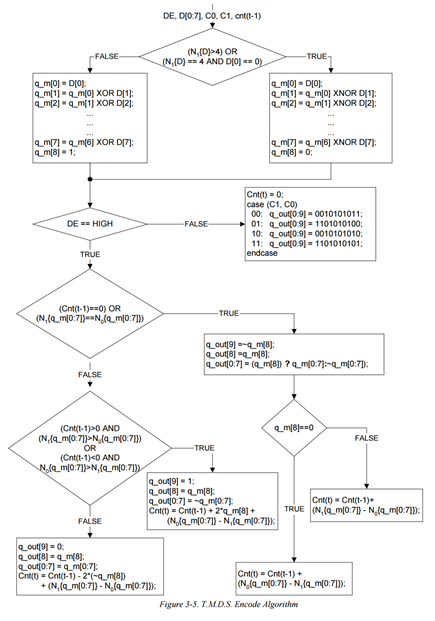TMDS comprises two different encoding schemes depending on whether pixel data or control data is being transmitted. This is similar to VGA, where we send control signals (HSYNC, VSYNC) in the blanking area of each frame. The pixel clock specification is calculated on the colour bit depth and resolution (how much data you need to send). As a general rule of thumb, this hasn’t changed since our last encounter with VGA, except now your TMDS clock will be 10x the speed of your base pixel clock.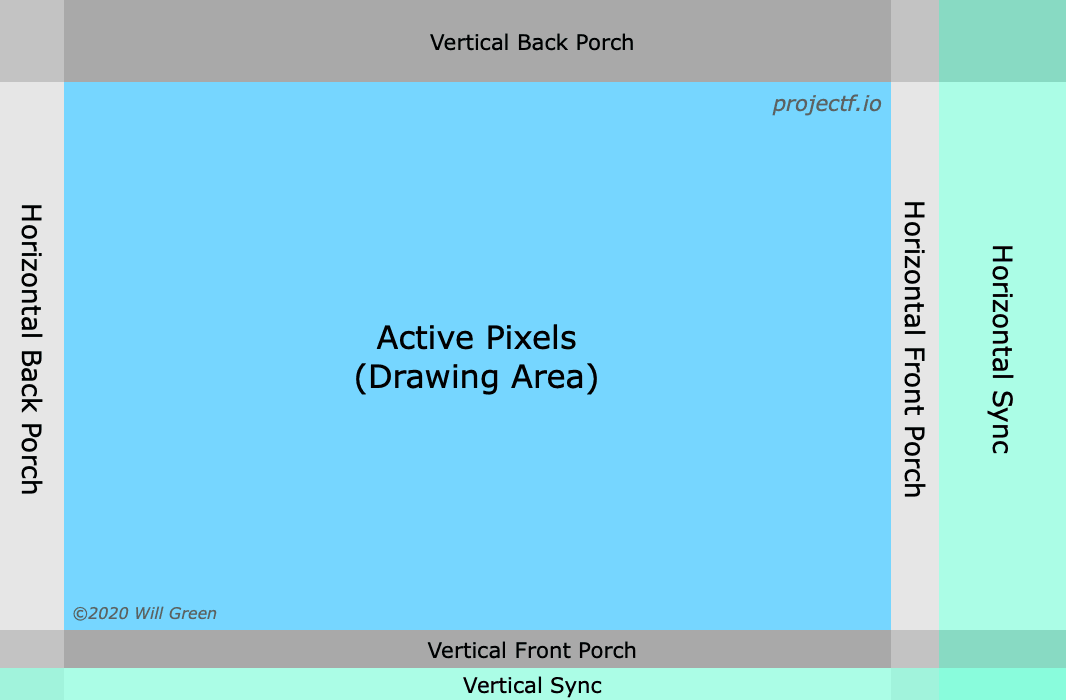##### Control Tokens

There are 4 10-bit control tokens used to transmit two bits of data. These are mapped to the HSYNC and VSYNC video signals, similar to VGA, and for synchronisation purposes. This is done in the blanking period. `c0` and `c1` represent the HSYNC and VSYNC signals respectively.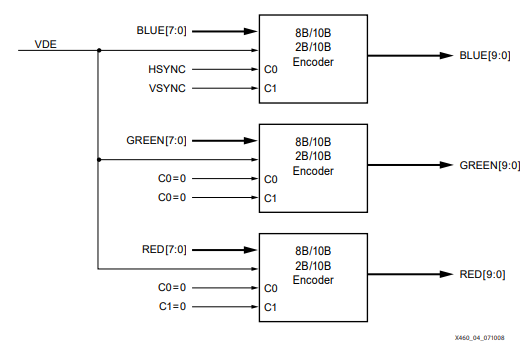The tokens are:

c0 c1 bits
0 0 10’b0010101011
0 1 10’b0010101010
1 0 10’b1101010100
1 1 10’b1101010101
##### Data Island (HDMI Only)

Additionally, HDMI defines a Data Island to transmit audio data and auxiliary data. This includes InfoFrames and other descriptive data, making it the key difference between the two protocols. This uses another encoding scheme called `TERC4` which allows 4 bits of data per channel to be sent.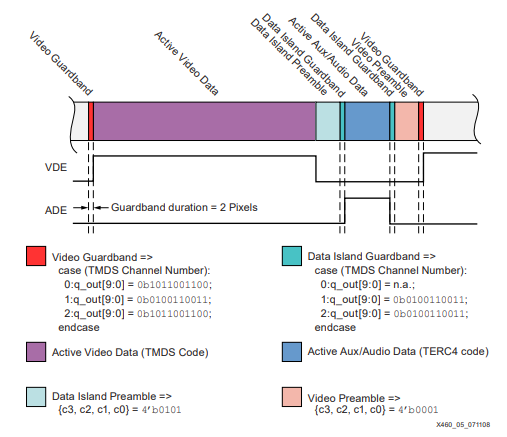``````case (D3, D2, D1, D0):
0000: q_out[9:0] = 0b1010011100;
0001: q_out[9:0] = 0b1001100011;
0010: q_out[9:0] = 0b1011100100;
0011: q_out[9:0] = 0b1011100010;
0100: q_out[9:0] = 0b0101110001;
0101: q_out[9:0] = 0b0100011110;
0110: q_out[9:0] = 0b0110001110;
0111: q_out[9:0] = 0b0100111100;
1000: q_out[9:0] = 0b1011001100;
1001: q_out[9:0] = 0b0100111001;
1010: q_out[9:0] = 0b0110011100;
1011: q_out[9:0] = 0b1011000110;
1100: q_out[9:0] = 0b1010001110;
1101: q_out[9:0] = 0b1001110001;
1110: q_out[9:0] = 0b0101100011;
1111: q_out[9:0] = 0b1011000011;
endcase;
``````
##### Pixel Data Encoding

To reduce the number of transitions in the data byte, TMDS uses `XOR` or `XNOR` encoding. Each bit is the XOR/XNOR of itself with the encoded version of the previous bit.

• Fewer than 4 ones, use `XOR`
• More than 4 ones, use `XNOR`
• Exactly 4 ones, start with 1 use `XOR`, start with 0 use `XNOR`

Add a 9th bit to describe the encoding method used, “1” for `XOR` and “0” for `XNOR`. This is likely the reason why a pixel clock is provided in DVI/HDMI, as opposed to VGA where the clock is recovered from the video data.

##### Maintaining DC Bias

The output of this encoding doesn’t guarantee an even number of ones and zeros, to create a net amplitude of zero. TMDS may invert a symbol and adds another bit to determine if the 10-bit symbol was inverted. This consists of the 8-bit data and 1-bit encoding, 1-bit inverted symbol.

### Verilog Implementation

This code is derived from fpga4fun’s post on HDMI.

We start by implementing the TMDS encoder for DVI, as mentioned above. To start off, we define the inputs/outputs to the module.

``````module TMDS_encoder(
input clk,
input [7:0] VD,  // video data (red, green or blue)
input [1:0] CD,  // control data
input VDE,  // video data enable, to choose between CD (when VDE=0) and VD (when VDE=1)
output reg [9:0] TMDS = 0
);
endmodule
``````

Next, we implement `XNOR` encoding the reduce the number of transitions in the data byte.

``````wire [3:0] Nb1s = VD + VD + VD + VD + VD + VD + VD + VD;
wire XNOR = (Nb1s>4'd4) || (Nb1s==4'd4 && VD==1'b0);
wire [8:0] q_m = {~XNOR, q_m[6:0] ^ VD[7:1] ^ {7{XNOR}}, VD};
``````

Then, we implement the code to generate the inverting bit, and invert the signal if necessary, for DC biasing.

``````reg [3:0] balance_acc = 0;
wire [3:0] balance = q_m + q_m + q_m + q_m + q_m + q_m + q_m + q_m - 4'd4;
wire balance_sign_eq = (balance == balance_acc);
wire invert_q_m = (balance==0 || balance_acc==0) ? ~q_m : balance_sign_eq;
wire [3:0] balance_acc_inc = balance - ({q_m ^ ~balance_sign_eq} & ~(balance==0 || balance_acc==0));
wire [3:0] balance_acc_new = invert_q_m ? balance_acc-balance_acc_inc : balance_acc+balance_acc_inc;
wire [9:0] TMDS_data = {invert_q_m, q_m, q_m[7:0] ^ {8{invert_q_m}}};
wire [9:0] TMDS_code = CD ? (CD ? 10'b1010101011 : 10'b0101010100) : (CD ? 10'b0010101011 : 10'b1101010100);
``````

Below is the full `TMDS_encoder` module.

``````module TMDS_encoder(
input clk,
input [7:0] VD,  // video data (red, green or blue)
input [1:0] CD,  // control data
input VDE,  // video data enable, to choose between CD (when VDE=0) and VD (when VDE=1)
output reg [9:0] TMDS = 0
);

wire [3:0] Nb1s = VD + VD + VD + VD + VD + VD + VD + VD;
wire XNOR = (Nb1s>4'd4) || (Nb1s==4'd4 && VD==1'b0);
wire [8:0] q_m = {~XNOR, q_m[6:0] ^ VD[7:1] ^ {7{XNOR}}, VD};

reg [3:0] balance_acc = 0;
wire [3:0] balance = q_m + q_m + q_m + q_m + q_m + q_m + q_m + q_m - 4'd4;
wire balance_sign_eq = (balance == balance_acc);
wire invert_q_m = (balance==0 || balance_acc==0) ? ~q_m : balance_sign_eq;
wire [3:0] balance_acc_inc = balance - ({q_m ^ ~balance_sign_eq} & ~(balance==0 || balance_acc==0));
wire [3:0] balance_acc_new = invert_q_m ? balance_acc-balance_acc_inc : balance_acc+balance_acc_inc;
wire [9:0] TMDS_data = {invert_q_m, q_m, q_m[7:0] ^ {8{invert_q_m}}};
wire [9:0] TMDS_code = CD ? (CD ? 10'b1010101011 : 10'b0101010100) : (CD ? 10'b0010101011 : 10'b1101010100);

always @(posedge clk) TMDS <= VDE ? TMDS_data : TMDS_code;
always @(posedge clk) balance_acc <= VDE ? balance_acc_new : 4'h0;
endmodule
``````

Next, we implement a simple top-level module with a test pattern generator. In this case, we use a 25MHz clock for the TMDS clock, and a 250MHz clock for the 10-bit data. To generate this clock frequency, we use a Phase Locked Loop (PLL) IP block. This allows us to use the PLL peripheral in the FPGA, which varies from vendor to vendor. Using the Tang Dynasty IDE, we can use the Tools > IP generator.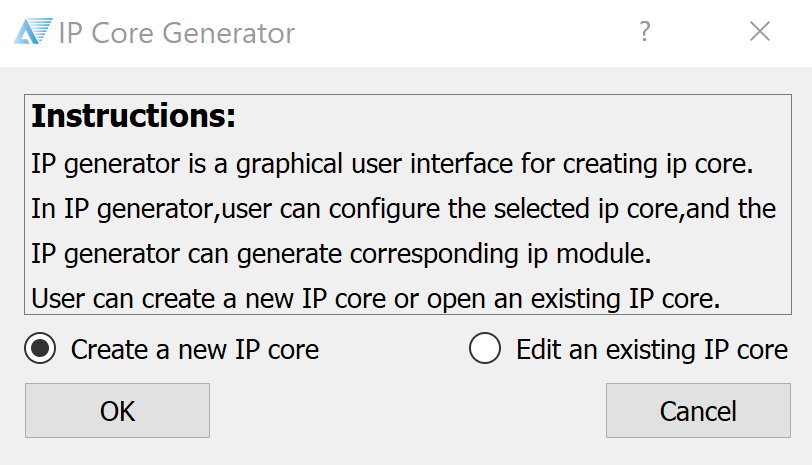Select “Create a new IP core”.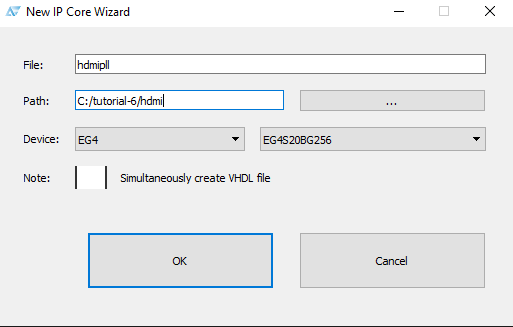Name your module, and make sure the correct device is selected for the Tang.Select the Phase Locked Loop (PLL) function.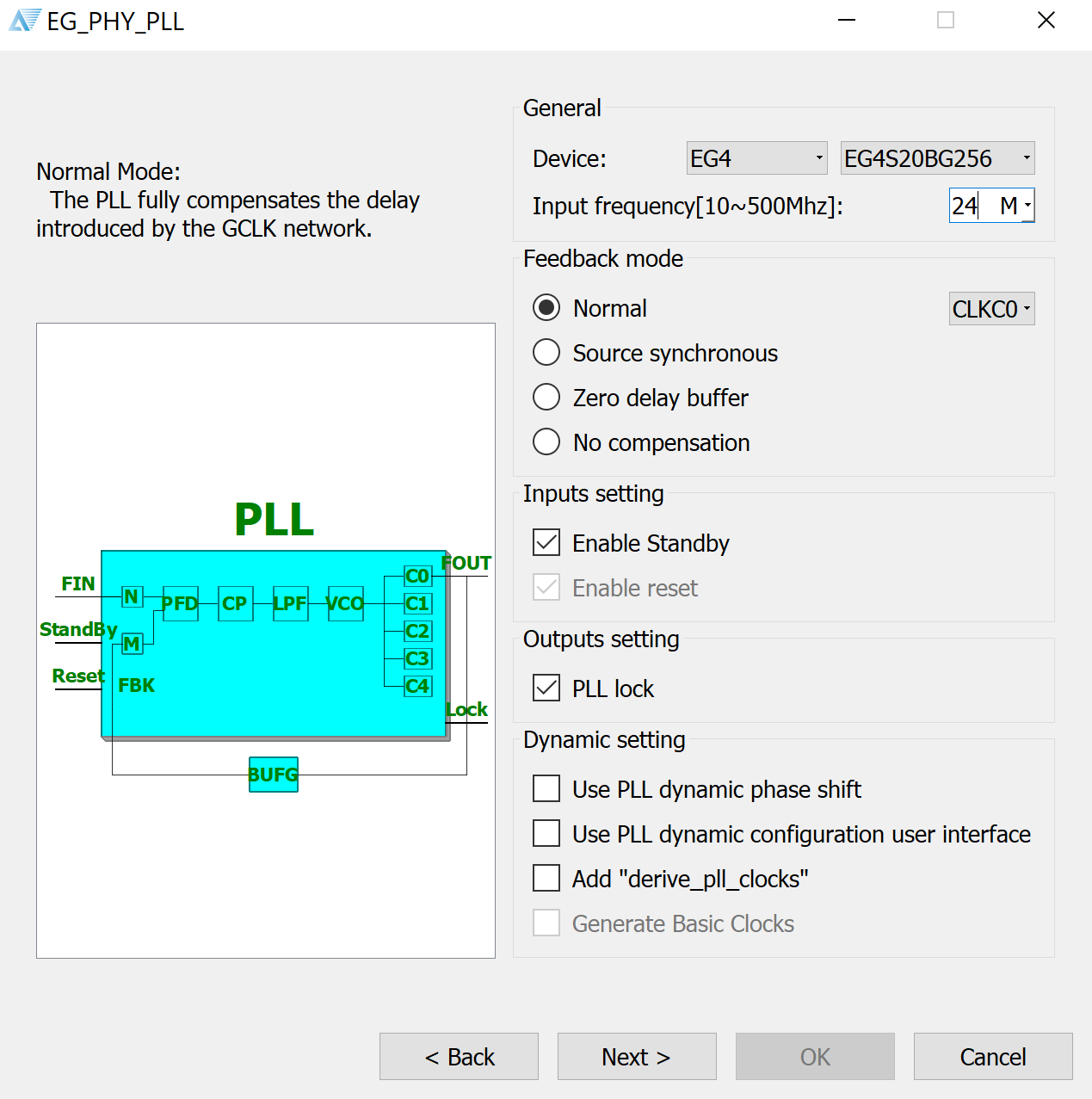Key in the input frequency of the external oscillator on the Tang, 24MHz.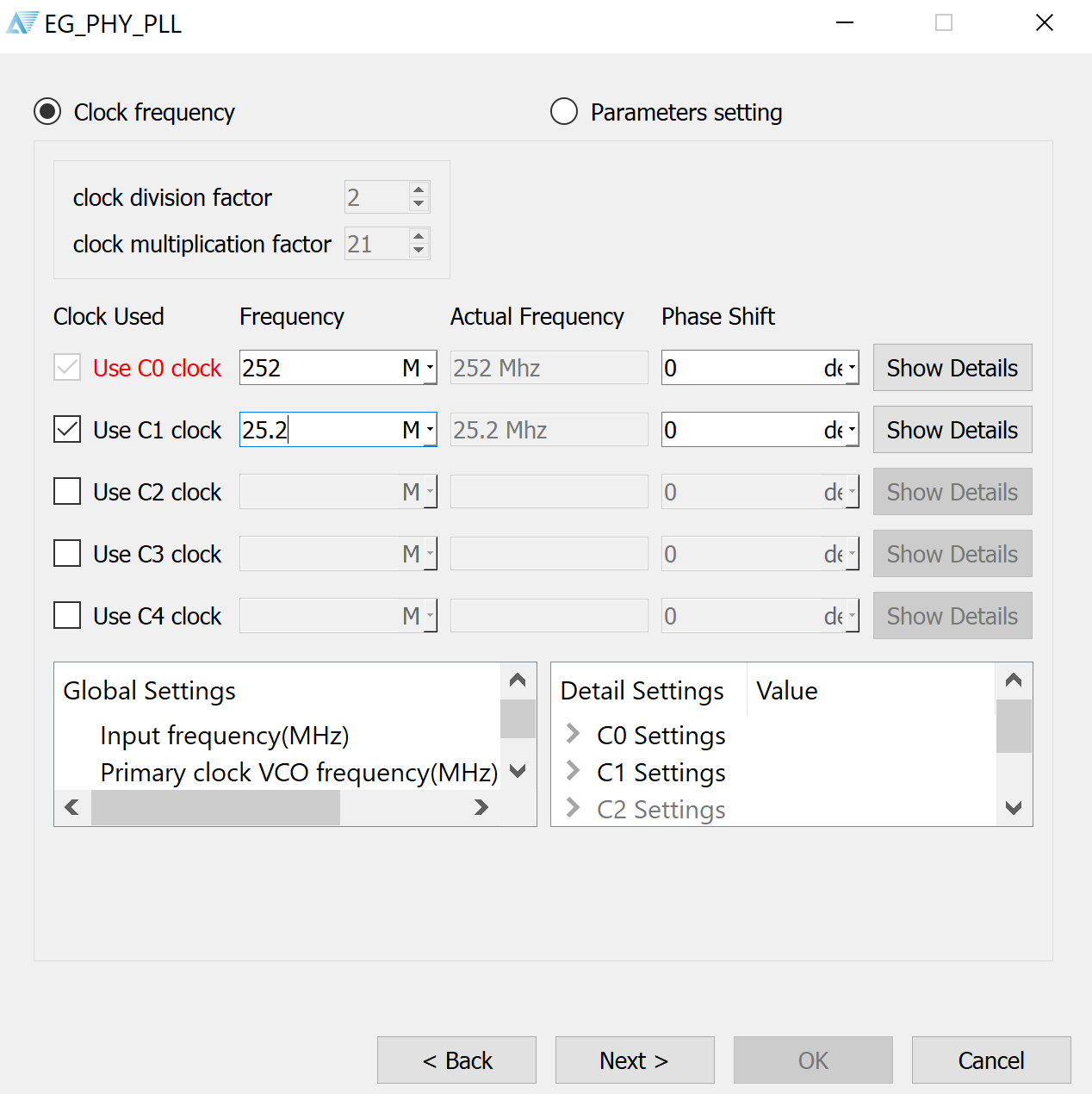Then, choose your desired output frequencies. For simplicity, we choose 252MHz and 25.2MHz, which is close enough to our desired frequencies of 25MHz pixel clock and 250MHz data frequency. We instantiate the PLL module as follows:

``````    pllhdmi pllInstance(.refclk(clk),
.reset(~rst),
.stdby(),
.extlock(),
.clk0_out(clk_TMDS),
.clk1_out(pixclk));
``````

Below is the full `HDMI_test` top level module. This includes a simple test pattern generator from fpga4fun.

``````module HDMI_test(
input clk,  // 24MHz
input rst,
output [2:0] TMDSp,
output TMDSp_clock,
output pixclk,
output HDMI_HPD
);

////////////////////////////////////////////////////////////////////////
wire clk_TMDS;
assign HDMI_HPD = 1'b1;

pllhdmi pllInstance(.refclk(clk),
.reset(~rst),
.stdby(),
.extlock(),
.clk0_out(clk_TMDS),
.clk1_out(pixclk));

////////////////////////////////////////////////////////////////////////
reg [9:0] CounterX, CounterY;
reg hSync, vSync, DrawArea;
always @(posedge pixclk) DrawArea <= (CounterX<640) && (CounterY<480);

always @(posedge pixclk) CounterX <= (CounterX==799) ? 0 : CounterX+1;
always @(posedge pixclk) if(CounterX==799) CounterY <= (CounterY==524) ? 0 : CounterY+1;

always @(posedge pixclk) hSync <= (CounterX>=656) && (CounterX<752);
always @(posedge pixclk) vSync <= (CounterY>=490) && (CounterY<492);

////////////////////////////////////////////////////////////////////////
wire [7:0] W = {8{CounterX[7:0]==CounterY[7:0]}};
wire [7:0] A = {8{CounterX[7:5]==3'h2 && CounterY[7:5]==3'h2}};
reg [7:0] red, green, blue;
always @(posedge pixclk) red <= ({CounterX[5:0] & {6{CounterY[4:3]==~CounterX[4:3]}}, 2'b00} | W) & ~A;
always @(posedge pixclk) green <= (CounterX[7:0] & {8{CounterY}} | W) & ~A;
always @(posedge pixclk) blue <= CounterY[7:0] | W | A;

////////////////////////////////////////////////////////////////////////
wire [9:0] TMDS_red, TMDS_green, TMDS_blue;
TMDS_encoder encode_R(.clk(pixclk), .VD(red  ), .CD(2'b00)        , .VDE(DrawArea), .TMDS(TMDS_red));
TMDS_encoder encode_G(.clk(pixclk), .VD(green), .CD(2'b00)        , .VDE(DrawArea), .TMDS(TMDS_green));
TMDS_encoder encode_B(.clk(pixclk), .VD(blue ), .CD({vSync,hSync}), .VDE(DrawArea), .TMDS(TMDS_blue));

////////////////////////////////////////////////////////////////////////
reg [3:0] TMDS_mod10=0;  // modulus 10 counter
reg [9:0] TMDS_shift_red=0, TMDS_shift_green=0, TMDS_shift_blue=0;
always @(posedge clk_TMDS) TMDS_shift_load <= (TMDS_mod10==4'd9);

always @(posedge clk_TMDS)
begin
TMDS_shift_red   <= TMDS_shift_load ? TMDS_red   : TMDS_shift_red  [9:1];
TMDS_shift_green <= TMDS_shift_load ? TMDS_green : TMDS_shift_green[9:1];
TMDS_shift_blue  <= TMDS_shift_load ? TMDS_blue  : TMDS_shift_blue [9:1];
TMDS_mod10 <= (TMDS_mod10==4'd9) ? 4'd0 : TMDS_mod10+4'd1;
end

assign TMDSp = TMDS_shift_red;
assign TMDSp = TMDS_shift_green;
assign TMDSp = TMDS_shift_blue;
assign TMDSp_clock = pixclk;
endmodule
``````

For implementation on our Lichee Tang FPGA, we have to define the differential pins for DVI output. I made a custom breakout to connect the pins on the 40P FPC connector to an HDMI port. The software automatically assigns the negative pair of the differential, based on the FPGA datasheet.

``````set_pin_assignment	{ TMDSp }	{ LOCATION = C1; IOSTANDARD = LVDS33; }
set_pin_assignment	{ TMDSp }	{ LOCATION = C3; IOSTANDARD = LVDS33; }
set_pin_assignment	{ TMDSp }	{ LOCATION = B2; IOSTANDARD = LVDS33; }
set_pin_assignment	{ TMDSp_clock }	{ LOCATION = E3; IOSTANDARD = LVDS33; }
set_pin_assignment	{ pixclk }	{ LOCATION = L1; IOSTANDARD = LVCMOS33; }
set_pin_assignment	{ HDMI_HPD }	{ LOCATION = G3; IOSTANDARD = LVCMOS33; }
set_pin_assignment	{ clk }	{ LOCATION = K14; }
set_pin_assignment  { rst } { LOCATION = K16; }
``````

#### Conclusion

For a deep dive into implementing your own DVI/HDMI module, take a look at this application note from Xilinx.

# Tutorial 5: VGA Display Output### VGA Display Output

VGA is an older display interface based on analog inputs of Red, Green and Blue for each pixel. The data for the pixels are changed by toggling `HSYNC` and `VSYNC` to indicate the active display area. The clock rate is defined by the resolution of the display output. A handy reference for VGA is available at Digikey. This tutorial is based on information from that blog post, as well as the relevant Nandland tutorial.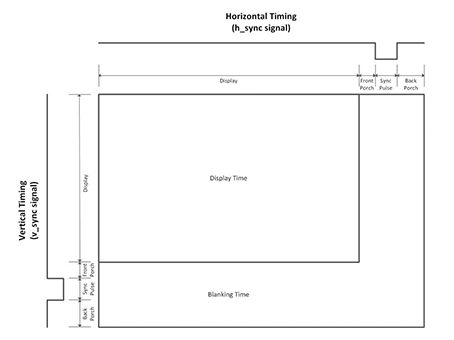Resolution Refresh Rate Pixel Clock (MHz) Display (H) Inactive Area (H) Display (V) Inactive Area (V)
640x480 60 25.175 640 160 480 120

From this, we know that we need to manage the VSYNC and HSYNC signals to control when we output RGB pixel data. Let’s start by creating a module that generates the appropriate VSYNC and HSYNC signals, given a certain fixed resolution. Create the following file `VGA_Sync_Pulses`.

``````// This module is designed for 640x480 with a 25 MHz input clock.

module VGA_Sync_Pulses
#(parameter TOTAL_COLS  = 800,
parameter TOTAL_ROWS  = 525,
parameter ACTIVE_COLS = 640,
parameter ACTIVE_ROWS = 480)
(input             i_Clk,
output            o_HSync,
output            o_VSync,
output reg [11:0] o_Col_Count = 0,
output reg [11:0] o_Row_Count = 0
);

always @(posedge i_Clk)
begin
if (o_Col_Count == TOTAL_COLS-1)
begin
o_Col_Count <= 0;
if (o_Row_Count == TOTAL_ROWS-1)
o_Row_Count <= 0;
else
o_Row_Count <= o_Row_Count + 1;
end
else
o_Col_Count <= o_Col_Count + 1;

end

// Only high in the ACTIVE AREA of the display
assign o_HSync = o_Col_Count < ACTIVE_COLS ? 1'b1 : 1'b0;
assign o_VSync = o_Row_Count < ACTIVE_ROWS ? 1'b1 : 1'b0;

endmodule
``````

The `always` block keeps track of the current pixel being described by the entire VGA module. This can be used from other modules to map graphics onto correct parts of the screen. The last blocks drive the HSYNC and VSYNC signals high when in the active area of the screen.

Next, let’s feed these signals into a test pattern generator (from Nandland). This module takes in the HSYNC and VSYNC signals and a pattern selector to generate the appropriate HSYNC, VSYNC and RGB signals.

``````// This module is designed for 640x480 with a 25 MHz input clock.
// All test patterns are being generated all the time.  This makes use of one
// of the benefits of FPGAs, they are highly parallelizable.  Many different
// things can all be happening at the same time.  In this case, there are several
// test patterns that are being generated simulatenously.  The actual choice of
// which test pattern gets displayed is done via the i_Pattern signal, which is
// an input to a case statement.

// Available Patterns:
// Pattern 0: Disables the Test Pattern Generator
// Pattern 1: All Red
// Pattern 2: All Green
// Pattern 3: All Blue
// Pattern 4: Checkerboard white/black
// Pattern 5: Color Bars
// Pattern 6: White Box with Border (2 pixels)

// Note: Comment out this line when building in iCEcube2:
`include "Sync_To_Count.v"

module Test_Pattern_Gen
#(parameter VIDEO_WIDTH = 3,
parameter TOTAL_COLS  = 800,
parameter TOTAL_ROWS  = 525,
parameter ACTIVE_COLS = 640,
parameter ACTIVE_ROWS = 480)
(input       i_Clk,
input [3:0] i_Pattern,
input       i_HSync,
input       i_VSync,
output reg  o_HSync = 0,
output reg  o_VSync = 0,
output reg [VIDEO_WIDTH-1:0] o_Red_Video,
output reg [VIDEO_WIDTH-1:0] o_Grn_Video,
output reg [VIDEO_WIDTH-1:0] o_Blu_Video);

wire w_VSync;
wire w_HSync;

// Patterns have 16 indexes (0 to 15) and can be g_Video_Width bits wide
wire [VIDEO_WIDTH-1:0] Pattern_Red[0:15];
wire [VIDEO_WIDTH-1:0] Pattern_Grn[0:15];
wire [VIDEO_WIDTH-1:0] Pattern_Blu[0:15];

// Make these unsigned counters (always positive)
wire [9:0] w_Col_Count;
wire [9:0] w_Row_Count;

wire [6:0] w_Bar_Width;
wire [2:0] w_Bar_Select;

Sync_To_Count #(.TOTAL_COLS(TOTAL_COLS),
.TOTAL_ROWS(TOTAL_ROWS))

UUT (.i_Clk      (i_Clk),
.i_HSync    (i_HSync),
.i_VSync    (i_VSync),
.o_HSync    (w_HSync),
.o_VSync    (w_VSync),
.o_Col_Count(w_Col_Count),
.o_Row_Count(w_Row_Count)
);

// Register syncs to align with output data.
always @(posedge i_Clk)
begin
o_VSync <= w_VSync;
o_HSync <= w_HSync;
end

/////////////////////////////////////////////////////////////////////////////
// Pattern 0: Disables the Test Pattern Generator
/////////////////////////////////////////////////////////////////////////////
assign Pattern_Red = 0;
assign Pattern_Grn = 0;
assign Pattern_Blu = 0;

/////////////////////////////////////////////////////////////////////////////
// Pattern 1: All Red
/////////////////////////////////////////////////////////////////////////////
assign Pattern_Red = (w_Col_Count < ACTIVE_COLS && w_Row_Count < ACTIVE_ROWS) ? {VIDEO_WIDTH{1'b1}} : 0;
assign Pattern_Grn = 0;
assign Pattern_Blu = 0;

/////////////////////////////////////////////////////////////////////////////
// Pattern 2: All Green
/////////////////////////////////////////////////////////////////////////////
assign Pattern_Red = 0;
assign Pattern_Grn = (w_Col_Count < ACTIVE_COLS && w_Row_Count < ACTIVE_ROWS) ? {VIDEO_WIDTH{1'b1}} : 0;
assign Pattern_Blu = 0;

/////////////////////////////////////////////////////////////////////////////
// Pattern 3: All Blue
/////////////////////////////////////////////////////////////////////////////
assign Pattern_Red = 0;
assign Pattern_Grn = 0;
assign Pattern_Blu = (w_Col_Count < ACTIVE_COLS && w_Row_Count < ACTIVE_ROWS) ? {VIDEO_WIDTH{1'b1}} : 0;

/////////////////////////////////////////////////////////////////////////////
// Pattern 4: Checkerboard white/black
/////////////////////////////////////////////////////////////////////////////
assign Pattern_Red = w_Col_Count ^ w_Row_Count ? {VIDEO_WIDTH{1'b1}} : 0;
assign Pattern_Grn = Pattern_Red;
assign Pattern_Blu = Pattern_Red;

/////////////////////////////////////////////////////////////////////////////
// Pattern 5: Color Bars
// Divides active area into 8 Equal Bars and colors them accordingly
// Colors Each According to this Truth Table:
// R G B  w_Bar_Select  Ouput Color
// 0 0 0       0        Black
// 0 0 1       1        Blue
// 0 1 0       2        Green
// 0 1 1       3        Turquoise
// 1 0 0       4        Red
// 1 0 1       5        Purple
// 1 1 0       6        Yellow
// 1 1 1       7        White
/////////////////////////////////////////////////////////////////////////////
assign w_Bar_Width = ACTIVE_COLS/8;

assign w_Bar_Select = w_Col_Count < w_Bar_Width*1 ? 0 :
w_Col_Count < w_Bar_Width*2 ? 1 :
w_Col_Count < w_Bar_Width*3 ? 2 :
w_Col_Count < w_Bar_Width*4 ? 3 :
w_Col_Count < w_Bar_Width*5 ? 4 :
w_Col_Count < w_Bar_Width*6 ? 5 :
w_Col_Count < w_Bar_Width*7 ? 6 : 7;

// Implement Truth Table above with Conditional Assignments
assign Pattern_Red = (w_Bar_Select == 4 || w_Bar_Select == 5 ||
w_Bar_Select == 6 || w_Bar_Select == 7) ?
{VIDEO_WIDTH{1'b1}} : 0;

assign Pattern_Grn = (w_Bar_Select == 2 || w_Bar_Select == 3 ||
w_Bar_Select == 6 || w_Bar_Select == 7) ?
{VIDEO_WIDTH{1'b1}} : 0;

assign Pattern_Blu = (w_Bar_Select == 1 || w_Bar_Select == 3 ||
w_Bar_Select == 5 || w_Bar_Select == 7) ?
{VIDEO_WIDTH{1'b1}} : 0;

/////////////////////////////////////////////////////////////////////////////
// Pattern 6: Black With White Border
// Creates a black screen with a white border 2 pixels wide around outside.
/////////////////////////////////////////////////////////////////////////////
assign Pattern_Red = (w_Row_Count <= 1 || w_Row_Count >= ACTIVE_ROWS-1-1 ||
w_Col_Count <= 1 || w_Col_Count >= ACTIVE_COLS-1-1) ?
{VIDEO_WIDTH{1'b1}} : 0;
assign Pattern_Grn = Pattern_Red;
assign Pattern_Blu = Pattern_Red;

/////////////////////////////////////////////////////////////////////////////
// Select between different test patterns
/////////////////////////////////////////////////////////////////////////////
always @(posedge i_Clk)
begin
case (i_Pattern)
4'h0 :
begin
o_Red_Video <= Pattern_Red;
o_Grn_Video <= Pattern_Grn;
o_Blu_Video <= Pattern_Blu;
end
4'h1 :
begin
o_Red_Video <= Pattern_Red;
o_Grn_Video <= Pattern_Grn;
o_Blu_Video <= Pattern_Blu;
end
4'h2 :
begin
o_Red_Video <= Pattern_Red;
o_Grn_Video <= Pattern_Grn;
o_Blu_Video <= Pattern_Blu;
end
4'h3 :
begin
o_Red_Video <= Pattern_Red;
o_Grn_Video <= Pattern_Grn;
o_Blu_Video <= Pattern_Blu;
end
4'h4 :
begin
o_Red_Video <= Pattern_Red;
o_Grn_Video <= Pattern_Grn;
o_Blu_Video <= Pattern_Blu;
end
4'h5 :
begin
o_Red_Video <= Pattern_Red;
o_Grn_Video <= Pattern_Grn;
o_Blu_Video <= Pattern_Blu;
end
4'h6 :
begin
o_Red_Video <= Pattern_Red;
o_Grn_Video <= Pattern_Grn;
o_Blu_Video <= Pattern_Blu;
end
default:
begin
o_Red_Video <= Pattern_Red;
o_Grn_Video <= Pattern_Grn;
o_Blu_Video <= Pattern_Blu;
end
endcase
end
endmodule
``````
``````// This module will take incoming horizontal and veritcal sync pulses and
// create Row and Column counters based on these syncs.
// It will align the Row/Col counters to the output Sync pulses.
// Useful for any module that needs to keep track of which Row/Col position we
// are on in the middle of a frame
module Sync_To_Count
#(parameter TOTAL_COLS = 800,
parameter TOTAL_ROWS = 525)
(input            i_Clk,
input            i_HSync,
input            i_VSync,
output reg       o_HSync = 0,
output reg       o_VSync = 0,
output reg [9:0] o_Col_Count = 0,
output reg [9:0] o_Row_Count = 0);

wire w_Frame_Start;

// Register syncs to align with output data.
always @(posedge i_Clk)
begin
o_VSync <= i_VSync;
o_HSync <= i_HSync;
end

// Keep track of Row/Column counters.
always @(posedge i_Clk)
begin
if (w_Frame_Start == 1'b1)
begin
o_Col_Count <= 0;
o_Row_Count <= 0;
end
else
begin
if (o_Col_Count == TOTAL_COLS-1)
begin
if (o_Row_Count == TOTAL_ROWS-1)
begin
o_Row_Count <= 0;
end
else
begin
o_Row_Count <= o_Row_Count + 1;
end
o_Col_Count <= 0;
end
else
begin
o_Col_Count <= o_Col_Count + 1;
end
end
end

// Look for rising edge on Vertical Sync to reset the counters
assign w_Frame_Start = (~o_VSync & i_VSync);

endmodule
``````

The test pattern generator chooses from a preset list of output patterns to drive the HSYNC, VSYNC and RGB lines. Additionally, the `Sync_To_Count` keeps track of the current pixel position in terms of Columns and Rows, an identical function to that in the `VGA_Sync_Pulses` module, as we didn’t use the outputs from that module. This was done just for convenience sake of keeping the `Test_Pattern_Generator` self-contained.

Lastly, we add the `VGA_Sync_Porch` module to add the inactive area to the output from the test pattern generator. Effectively, this modifies the HSYNC and VSYNC signals when in the inactive area to include the front porch and back porch, where they should be driven high.

``````// The purpose of this module is to modify the input HSync and VSync signals to
// include some time for what is called the Front and Back porch.  The front
// and back porch of a VGA interface used to have more meaning when a monitor
// actually used a Cathode Ray Tube (CRT) to draw an image on the screen.  You
// can read more about the details of how old VGA monitors worked.  These
// days, the notion of a front and back porch is maintained, due more to
// convention than to the physics of the monitor.
// New standards like DVI and HDMI which are meant for digital signals have
// removed this notion of the front and back porches.  Remember that VGA is an
// This module is designed for 640x480 with a 25 MHz input clock.

module VGA_Sync_Porch #(parameter VIDEO_WIDTH = 3,  // remember to
parameter TOTAL_COLS  = 3,  // overwrite
parameter TOTAL_ROWS  = 3,  // these defaults
parameter ACTIVE_COLS = 2,
parameter ACTIVE_ROWS = 2)
(input i_Clk,
input i_HSync,
input i_VSync,
input [VIDEO_WIDTH-1:0] i_Red_Video,
input [VIDEO_WIDTH-1:0] i_Grn_Video,
input [VIDEO_WIDTH-1:0] i_Blu_Video,
output reg o_HSync,
output reg o_VSync,
output reg [VIDEO_WIDTH-1:0] o_Red_Video,
output reg [VIDEO_WIDTH-1:0] o_Grn_Video,
output reg [VIDEO_WIDTH-1:0] o_Blu_Video
);

parameter c_FRONT_PORCH_HORZ = 18;
parameter c_BACK_PORCH_HORZ  = 50;
parameter c_FRONT_PORCH_VERT = 10;
parameter c_BACK_PORCH_VERT  = 33;

wire w_HSync;
wire w_VSync;

wire [9:0] w_Col_Count;
wire [9:0] w_Row_Count;

reg [VIDEO_WIDTH-1:0] r_Red_Video = 0;
reg [VIDEO_WIDTH-1:0] r_Grn_Video = 0;
reg [VIDEO_WIDTH-1:0] r_Blu_Video = 0;

Sync_To_Count #(.TOTAL_COLS(TOTAL_COLS),
.TOTAL_ROWS(TOTAL_ROWS)) UUT
(.i_Clk      (i_Clk),
.i_HSync    (i_HSync),
.i_VSync    (i_VSync),
.o_HSync    (w_HSync),
.o_VSync    (w_VSync),
.o_Col_Count(w_Col_Count),
.o_Row_Count(w_Row_Count)
);

// Purpose: Modifies the HSync and VSync signals to include Front/Back Porch
always @(posedge i_Clk)
begin
if ((w_Col_Count < c_FRONT_PORCH_HORZ + ACTIVE_COLS) ||
(w_Col_Count > TOTAL_COLS - c_BACK_PORCH_HORZ - 1))
o_HSync <= 1'b1;
else
o_HSync <= w_HSync;

if ((w_Row_Count < c_FRONT_PORCH_VERT + ACTIVE_ROWS) ||
(w_Row_Count > TOTAL_ROWS - c_BACK_PORCH_VERT - 1))
o_VSync <= 1'b1;
else
o_VSync <= w_VSync;
end

// Purpose: Align input video to modified Sync pulses.
// Adds in 2 Clock Cycles of Delay
always @(posedge i_Clk)
begin
r_Red_Video <= i_Red_Video;
r_Grn_Video <= i_Grn_Video;
r_Blu_Video <= i_Blu_Video;

o_Red_Video <= r_Red_Video;
o_Grn_Video <= r_Grn_Video;
o_Blu_Video <= r_Blu_Video;
end

endmodule
``````

Lastly, we combine all of the above modules into a top level file called `vga_top.v`.

``````module vga_top
#(
parameter c_TOTAL_COLS 			= 800,
parameter c_TOTAL_ROWS    		= 525,
parameter c_ACTIVE_COLS			= 640,
parameter c_ACTIVE_ROWS 		= 480,
parameter c_VIDEO_WIDTH 		= 3 // 3 bits per pixel
)

(
// 24MHz clock on board
input wire clk,
input wire rst,

// VGA Connections
output wire [c_VIDEO_WIDTH-1:0] R,
output wire [c_VIDEO_WIDTH-1:0] G,
output wire [c_VIDEO_WIDTH-1:0] B,
output wire o_VGA_HSync,
output wire o_VGA_VSync
);

// Internal R,G,B wires: VGA Signals
wire [c_VIDEO_WIDTH-1:0] w_Red_Video_TP, w_Red_Video_Porch;
wire [c_VIDEO_WIDTH-1:0] w_Grn_Video_TP, w_Grn_Video_Porch;
wire [c_VIDEO_WIDTH-1:0] w_Blu_Video_TP, w_Blu_Video_Porch;

// VGA_Sync_Pulses to generate HSYNC and VSYNC
VGA_Sync_Pulses   #(
.TOTAL_COLS  (c_TOTAL_COLS),
.TOTAL_ROWS  (c_TOTAL_ROWS),
.ACTIVE_COLS (c_ACTIVE_COLS),
.ACTIVE_ROWS (c_ACTIVE_ROWS)
) VGA_Sync_Pulses_Inst (
.i_Clk (clk),
.o_HSync (w_HSync_Start),
.o_VSync (w_VSync_Start),
.o_Col_Count (),
.o_Row_Count ()
);

// Test pattern to generate R,G,B signals
Test_Pattern_Gen  #(
.VIDEO_WIDTH(c_VIDEO_WIDTH),
.TOTAL_COLS(c_TOTAL_COLS),
.TOTAL_ROWS(c_TOTAL_ROWS),
.ACTIVE_COLS(c_ACTIVE_COLS),
.ACTIVE_ROWS(c_ACTIVE_ROWS))
Test_Pattern_Gen_Inst(
.i_Clk(clk),
.i_Pattern(4'h1), // color bars
.i_HSync(w_HSync_Start),
.i_VSync(w_VSync_Start),
.o_HSync(w_HSync_TP),
.o_VSync(w_VSync_TP),
.o_Red_Video(w_Red_Video_TP),
.o_Grn_Video(w_Grn_Video_TP),
.o_Blu_Video(w_Blu_Video_TP));

// Add inactive area to output HSYNC, VSYNC from test pattern
VGA_Sync_Porch  #(
.VIDEO_WIDTH(c_VIDEO_WIDTH),
.TOTAL_COLS(c_TOTAL_COLS),
.TOTAL_ROWS(c_TOTAL_ROWS),
.ACTIVE_COLS(c_ACTIVE_COLS),
.ACTIVE_ROWS(c_ACTIVE_ROWS))
VGA_Sync_Porch_Inst(
.i_Clk(clk),
.i_HSync(w_HSync_TP),
.i_VSync(w_VSync_TP),
.i_Red_Video(w_Red_Video_TP),
.i_Grn_Video(w_Grn_Video_TP),
.i_Blu_Video(w_Blu_Video_TP),
.o_HSync(w_HSync_Porch),
.o_VSync(w_VSync_Porch),
.o_Red_Video(w_Red_Video_Porch),
.o_Grn_Video(w_Grn_Video_Porch),
.o_Blu_Video(w_Blu_Video_Porch));

// Send final signals to output pins
assign o_VGA_HSync = w_HSync_Porch;
assign o_VGA_VSync = w_VSync_Porch;

assign R = w_Red_Video_Porch;
assign G = w_Grn_Video_Porch;
assign B = w_Blu_Video_Porch;

endmodule
``````

In this module, we set the resolution parameters for the modules and use the defaults for the front porch, back porch and sync pulse in the inactive area. Then, we define the wires connecting the modules together, and to external components such as the onboard 24 MHz clock, the reset button (active low) and the VGA connector. The 24 MHz clock isn’t optimal as a 25.175MHz clock is specified. However, it’s good enough for our learning purposes. Optionally, you can use the PLL IP module to generate the correct clock, but it varies from vendor to vendor and its code isn’t transferrable across vendors.

In my case, I use the VGA PS2 board from Waveshare, which uses an R2R DAC to generate 3-bit RGB colour. 3V3 logic levels on the FPGA result in a maximum 0.7V analog voltage to the VGA connector, the maximum value for each colour.

With this, congratulations! You’ve made your first video output with VGA and are on track to do great things with FPGAs! By now you should be sufficiently well versed in Verilog and are able to understand how HDL code is designed and executed. I recommend trying out Nandland’s Pong walkthrough or running the PicoRV32 core on this FPGA as a stretcher exercise. The possibilities are endless!

# Tutorial 4: FIFO Buffer### FIFO Buffer

In our previous tutorial, our UART interface could only send and receive one byte at a time. To solve that problem, let’s implement a First In First Out (FIFO) buffer to hold the previous two values in data registers. We define some specifications for our FIFO buffer below.

• 16-bit data bus
• Full and Empty flags
• Overflow and underflow flags

We start by defining the ports to our module.

``````module fifo_memory (
input i_Clock,
input i_Reset,
input i_Write_En,
input  [c_WIDTH:0] i_Data_In,
output [c_WIDTH:0] o_Data_Out,
output reg fifo_full,
output reg fifo_empty,
output reg fifo_overflow,
output reg fifo_underflow
);
endmodule
``````

Then, we define the internal signals we use to store and access the memory.

``````    // Internal memory, 7 16-bit wide registers
parameter c_DEPTH = 7;
parameter c_WIDTH = 15;
reg [c_WIDTH:0] memory [0:c_DEPTH];
reg [c_WIDTH:0] r_Data_Out;
``````

Then, we define logic for reading from and writing to the internal memory of the FIFO buffer, sequentially.

``````    // Writing to FIFO
always @(posedge i_Clock) begin
if (i_Write_En) begin

fifo_overflow <= 1'b0;
end
else
fifo_overflow <= 1'b1;
end
end

always @(posedge i_Clock) begin

if (!fifo_empty) begin
fifo_underflow <= 1'b0;
end
else
fifo_underflow <= 1'b1;
end
end

assign o_Data_Out = r_Data_Out;
``````

Next, we want to manage the `fifo-full` and `fifo-empty` flags that we use to guide read and write operations. This section is referenced from zipcpu, as it provides an efficient way to set read and write flags in one clock cycle.

``````
// Calculating full/empty flags, referenced from zipcpu.com
assign	dblnext = wraddr + 2;

always @(posedge i_Clock)
if (!i_Reset)
begin
fifo_full <= 1'b0;
fifo_empty <= 1'b1;
end else casez({ i_Write_En, i_Read_En, !fifo_full, !fifo_empty })
4'b01?1: begin	// A successful read
fifo_full  <= 1'b0;
end
4'b101?: begin	// A successful write
fifo_empty <= 1'b0;
end
4'b11?0: begin	// Successful write, failed read
fifo_full  <= 1'b0;
fifo_empty <= 1'b0;
end
4'b11?1: begin	// Successful read and write
fifo_full  <= fifo_full;
fifo_empty <= 1'b0;
end
default: begin end
endcase
``````

Lastly, we bring it all together for the final file `fifo_memory.v`.

``````module fifo_memory (
input i_Clock,
input i_Reset,
input i_Write_En,
input  [c_WIDTH:0] i_Data_In,
output [c_WIDTH:0] o_Data_Out,
output reg fifo_full,
output reg fifo_empty,
output reg fifo_overflow,
output reg fifo_underflow
);

// Internal memory, 7 16-bit wide registers
parameter c_DEPTH = 7;
parameter c_WIDTH = 7;
reg [c_WIDTH:0] memory [0:c_DEPTH];
reg [c_WIDTH:0] r_Data_Out;

// Writing to FIFO
always @(posedge i_Clock) begin
if (i_Write_En) begin

fifo_overflow <= 1'b0;
end
else
fifo_overflow <= 1'b1;
end
end

always @(posedge i_Clock) begin

if (!fifo_empty) begin
fifo_underflow <= 1'b0;
end
else
fifo_underflow <= 1'b1;
end
end

assign o_Data_Out = r_Data_Out;

// Calculating full/empty flags, referenced from zipcpu.com
assign	dblnext = wraddr + 2;

always @(posedge i_Clock, negedge i_Reset)

// Reset case
if (!i_Reset)
begin
// Reset output flags
fifo_full <= 1'b0;
fifo_empty <= 1'b1;

end else casez({ i_Write_En, i_Read_En, !fifo_full, !fifo_empty })
4'b01?1: begin	// A successful read
fifo_full  <= 1'b0;
end
4'b101?: begin	// A successful write
fifo_empty <= 1'b0;
end
4'b11?0: begin	// Successful write, failed read
fifo_full  <= 1'b0;
fifo_empty <= 1'b0;
end
4'b11?1: begin	// Successful read and write
fifo_full  <= fifo_full;
fifo_empty <= 1'b0;
end
default: begin end
endcase

endmodule
``````

Let’s write a testbench to validate the output signals of our module. By now you should be familiar with the general structure of a testbench.

1. Describe test signals
2. Instantiate unit under test (can be multiple of them)
3. Put testbench logic under `initial` block to run once. Use `always` block for repeating logic
4. Use `if` statements to validate outputs and print outputs using `\$display()`
5. Save output waveform using `\$dumpfile()` and `\$dumpvars()`
```````timescale 1ns/1ns
`include "fifo_memory.v"

module fifo_memory_tb ();

// Test signals
reg r_Clock = 0;
reg r_Reset = 1;
reg r_Write_En = 0;
reg  [15:0] r_Data_In = 0;
wire [15:0] w_Data_Out;
wire w_fifo_full;
wire w_fifo_empty;
wire w_fifo_overflow;
wire w_fifo_underflow;

parameter c_CLOCK_PERIOD_NS = 10;

// Instantiate module
fifo_memory #(
.c_DEPTH(7),
.c_WIDTH(15)
) UUT (
.i_Clock(r_Clock),
.i_Reset(r_Reset),
.i_Write_En(r_Write_En),
.i_Data_In(r_Data_In),
.o_Data_Out(w_Data_Out),
.fifo_full(w_fifo_full),
.fifo_empty(w_fifo_empty),
.fifo_overflow(w_fifo_overflow),
.fifo_underflow(w_fifo_underflow)
);

// Testbench logic
always
#(c_CLOCK_PERIOD_NS/2) r_Clock <= !r_Clock;

// Main Testing:
initial
begin
// Initialise module through reset
r_Reset = ~r_Reset;
#10
r_Reset = ~r_Reset;
#10

// Write two bytes
r_Data_In  <= 16'hBEEF;
r_Write_En <= 1'b1;
#10;
r_Write_En <= 1'b0;
#10
// Check that the correct data was received
if (w_Data_Out == 16'hBEEF)
\$display("Test Passed - Correct two bytes received");
else
\$display("Test Failed - Incorrect two bytes received");

// Try overflowing it
r_Write_En <= 1'b0;

for (integer i = 16'h0; i < 16'h1FF; i = i + 1'b1) begin
r_Data_In  <= i;
r_Write_En <= 1'b1;
#10;
end
r_Write_En <= 1'b0;
if (w_fifo_overflow)
\$display("Test Passed - Overflow flag works");
else
\$display("Test Failed - Overflow flag failed");

// Try underflowing it
r_Write_En <= 1'b0;

for (integer i = 16'h0; i < 16'h2FF; i = i + 1'b1) begin
#10;
end
r_Write_En <= 1'b0;
if (w_fifo_underflow)
\$display("Test Passed - Underflow flag works");
else
\$display("Test Failed - Underflow flag failed");
\$finish();
end

initial
begin
// Required to dump signals
\$dumpfile("dump.vcd");
\$dumpvars(0);
end

endmodule
``````

Running the simulation in `iverilog` and viewing in `gtkwave` gives the following result.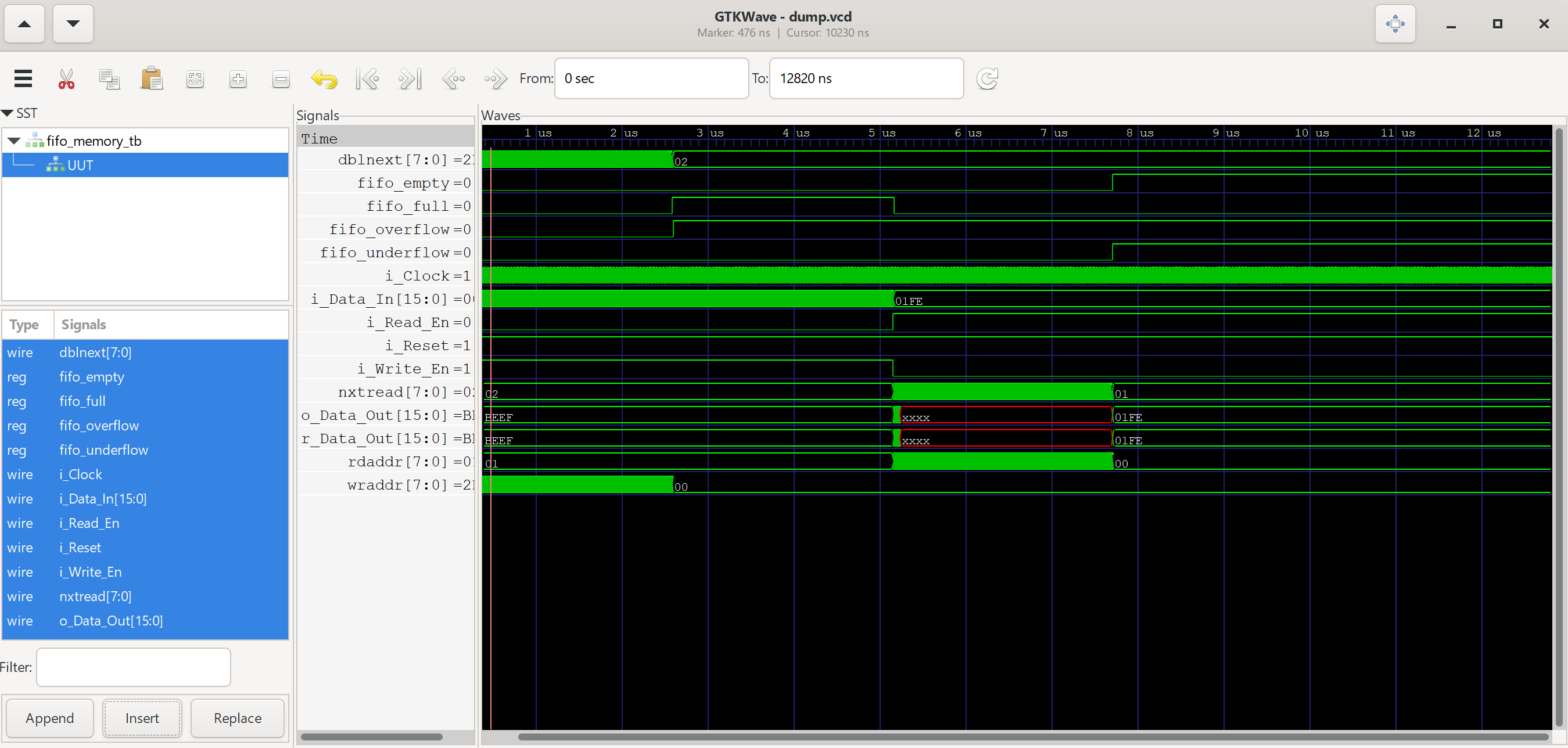Congratulations! You’ve defined your first FIFO buffer. In practice, FIFO buffers are very useful for the following situations.

• Crossing clock domains
• Buffering high speed, infrequent data
• Aligning data for math operations
• Buffering data coming from software, to be sent out of the chip

Now, let’s use this for our UART peripheral we designed in Tutorial 3!

#### Complete UART Transceiver

Let’s draw up a block diagram of what our UART transceiver should look like.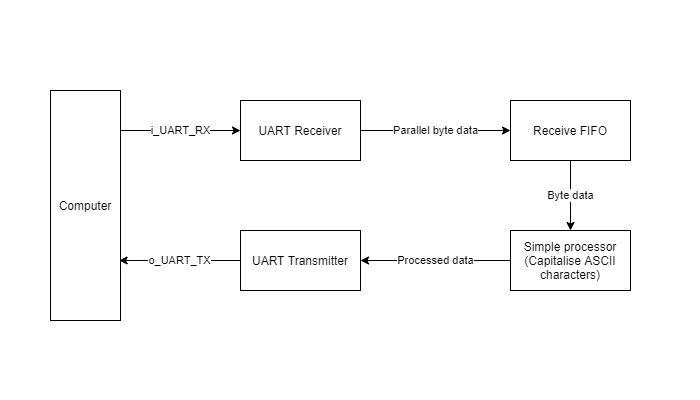We’ll connect the RX, TX and FIFO modules that we’ve already created in this fashion. For some simple processing, we’ll include a module that converts lower case characters to upper case characters, using a simple arithmetic operation (`-32` to convert from lower to upper case).

Let’s start by designing the missing module to convert from lower to upper case. Hopefully, you’ve gotten the hang of Verilog from previous tutorials and are able to understand what this does!

``````module lower_to_upper (
input i_Clock,
input i_Reset,
input i_Data_Empty,
input [7:0] i_data,
output [7:0] o_data,
output o_write_enable,
);

// Internal registers

// Shift register to delay one clock cycle
always @(posedge i_Clock) begin
end

// Outputs
assign o_write_enable = ~i_Data_Empty;
assign o_data = i_data - 8'h20;

endmodule
``````

Let’s write a simple testbench for this module.

```````timescale 1ps/1ps
`include "lower_to_upper.v"

module lower_to_upper_tb ();

reg r_Clock = 0;
reg r_Reset = 1;
reg r_Data_Empty = 1;
reg [7:0] r_data = 8'b0;
wire [7:0] w_data_out;
wire w_write_enable;

parameter c_CLOCK_PERIOD_NS = 10;

lower_to_upper UUT (
.i_Clock(r_Clock),
.i_Reset(r_Reset),
.i_Data_Empty(r_Data_Empty),
.i_data(r_data),
.o_data(w_data_out),
.o_write_enable(w_write_enable),
);

always #(c_CLOCK_PERIOD_NS/2) r_Clock <= !r_Clock;

initial begin
r_Data_Empty <= 0;
r_data <= 8'h61;
#(c_CLOCK_PERIOD_NS)

if (w_data_out == 8'h41)
\$display("Test passed");
else
\$display("Test failed, 0x%0h",w_data_out);

\$finish();

end

initial
begin
// Required to dump signals
\$dumpfile("dump.vcd");
\$dumpvars(0);
end

endmodule
``````

Next, let’s combine everything together in a top-level module.

```````include "UART_RX.v"
`include "UART_TX.v"
`include "fifo_memory.v"
`include "lower_to_upper.v"

module UART_Transceiver (
input i_Clock,
input i_Reset,
input i_RX_Serial,
output o_TX_Serial,
output o_TX_Done
);

// Board uses a 24 MHz clock
// Want to interface to 115200 baud UART
// 24000000 / 115200 = 208 Clocks Per Bit.
parameter CLOCK_PERIOD_NS = 41;
parameter CLKS_PER_BIT    = 208;
parameter BIT_PERIOD      = 8600;
parameter FIFO_DEPTH      = 7;
parameter FIFO_WIDTH      = 7;

// UART RX signals
wire UART_RX_Data_Valid;
wire [7:0] UART_RX_Byte;

// UART_RX instance
UART_RX #(.CLKS_PER_BIT(CLKS_PER_BIT)) UART_RX_INST
(.i_Clock(i_Clock),
.i_Reset(i_Reset),
.i_RX_Serial(i_RX_Serial),
.o_RX_Data_Valid(UART_RX_Data_Valid),
.o_RX_Byte(UART_RX_Byte)
);

// FIFO signals
wire fifo_Write_En;
wire [7:0] fifo_Data_In;
wire [7:0] fifo_Data_Out;
wire fifo_full;
wire fifo_empty;
wire fifo_overflow;
wire fifo_underflow;

assign fifo_Data_In  = UART_RX_Byte;
assign fifo_Write_En = UART_RX_Data_Valid ? 1'b1 : 1'b0;

// FIFO instance
fifo_memory #(
.c_DEPTH(FIFO_DEPTH),
.c_WIDTH(FIFO_WIDTH)
) fifo_memory_instance (
.i_Clock(i_Clock),
.i_Reset(i_Reset),
.i_Write_En(fifo_Write_En),
.i_Data_In(fifo_Data_In),
.o_Data_Out(fifo_Data_Out),
.fifo_full(fifo_full),
.fifo_empty(fifo_empty),
.fifo_overflow(fifo_overflow),
.fifo_underflow(fifo_underflow)
);

// Lower to Upper signals
wire [7:0] l2u_data_in;
wire [7:0] l2u_data_out;
wire l2u_write_enable;

assign l2u_data_in      = fifo_Data_Out;

// Lower to Upper instance
lower_to_upper lower_to_upper_instance(
.i_Clock(i_Clock),
.i_Reset(i_Reset),
.i_Data_Empty(fifo_empty),
.i_data(l2u_data_in),
.o_data(l2u_data_out),
.o_write_enable(l2u_write_enable),
);

// UART TX signals
wire UART_TX_DV;
wire [7:0] UART_TX_Byte;
wire UART_TX_Active;

assign UART_TX_Byte = l2u_data_out;

// UART TX instance
UART_TX #(
.CLKS_PER_BIT(CLKS_PER_BIT)
) UART_TX_Inst (
.i_Clock(i_Clock),
.i_Reset(i_Reset),
.i_TX_DV(UART_TX_DV),
.i_TX_Byte(UART_TX_Byte),
.o_TX_Active(UART_TX_Active),
.o_TX_Serial(o_TX_Serial),
.o_TX_Done(o_TX_Done)
);

endmodule
``````

Next, we write a testbench to send an ASCII character through UART, then receive the output through UART.

```````include "UART_Transceiver.v"
`timescale 1ps/1ps

module UART_Transceiver_tb();
// Test signals
reg r_Clock;
reg r_Reset;
reg r_RX_Serial;
wire w_TX_Serial;
wire w_TX_Done;

// Testbench signals
wire w_RX_Byte;
reg  r_RX_Byte;

parameter c_CLOCK_PERIOD_NS = 40; //40
parameter c_CLKS_PER_BIT    = 208; //208
parameter c_BIT_PERIOD      = 8600; //8600
parameter c_FIFO_DEPTH      = 7;
parameter c_FIFO_WIDTH      = 7;

// Instantiate top module
UART_Transceiver UUT (
.i_Clock(r_Clock),
.i_Reset(r_Reset),
.i_RX_Serial(r_RX_Serial),
.o_TX_Serial(w_TX_Serial),
.o_TX_Done(w_TX_Done)
);

// Takes in input byte and serializes it
input [7:0] i_Data;
integer     ii;
begin
// Send Start Bit
r_RX_Serial <= 1'b0;
#(c_BIT_PERIOD);
#(c_BIT_PERIOD/8);

// Send Data Byte
for (ii=0; ii<8; ii=ii+1)
begin
r_RX_Serial <= i_Data[ii];
#(c_BIT_PERIOD);
end

// Send Stop Bit
r_RX_Serial <= 1'b1;
#(c_BIT_PERIOD);
end

// Takes in input UART and deserializes it
integer     iii;
begin
// #(c_BIT_PERIOD);
#1000;

for (iii=0; iii<8; iii=iii+1)
begin
#(c_BIT_PERIOD);
end

#(c_BIT_PERIOD);
end

always #(c_CLOCK_PERIOD_NS/2) r_Clock <= !r_Clock;

initial begin
r_RX_Serial = 1;
r_Reset = 1;
r_Clock = 0;

// Initialise module through reset
r_Reset = ~r_Reset;
@(posedge r_Clock);
r_Reset = ~r_Reset;
@(posedge r_Clock);

// Send a command to the UART (exercise Rx)
@(posedge r_Clock);
UART_WRITE_BYTE(8'h61); // 'a' in ASCII
@(posedge r_Clock);

// Check that the correct command was received
@(posedge r_Clock);
@(posedge r_Clock);
\$display("Test Passed - Correct Byte Received");
else

\$finish();
end

initial
begin
// Required to dump signals
\$dumpfile("dump.vcd");
\$dumpvars(0);
end

endmodule
``````

Running this through `gtkwave` gives the following output.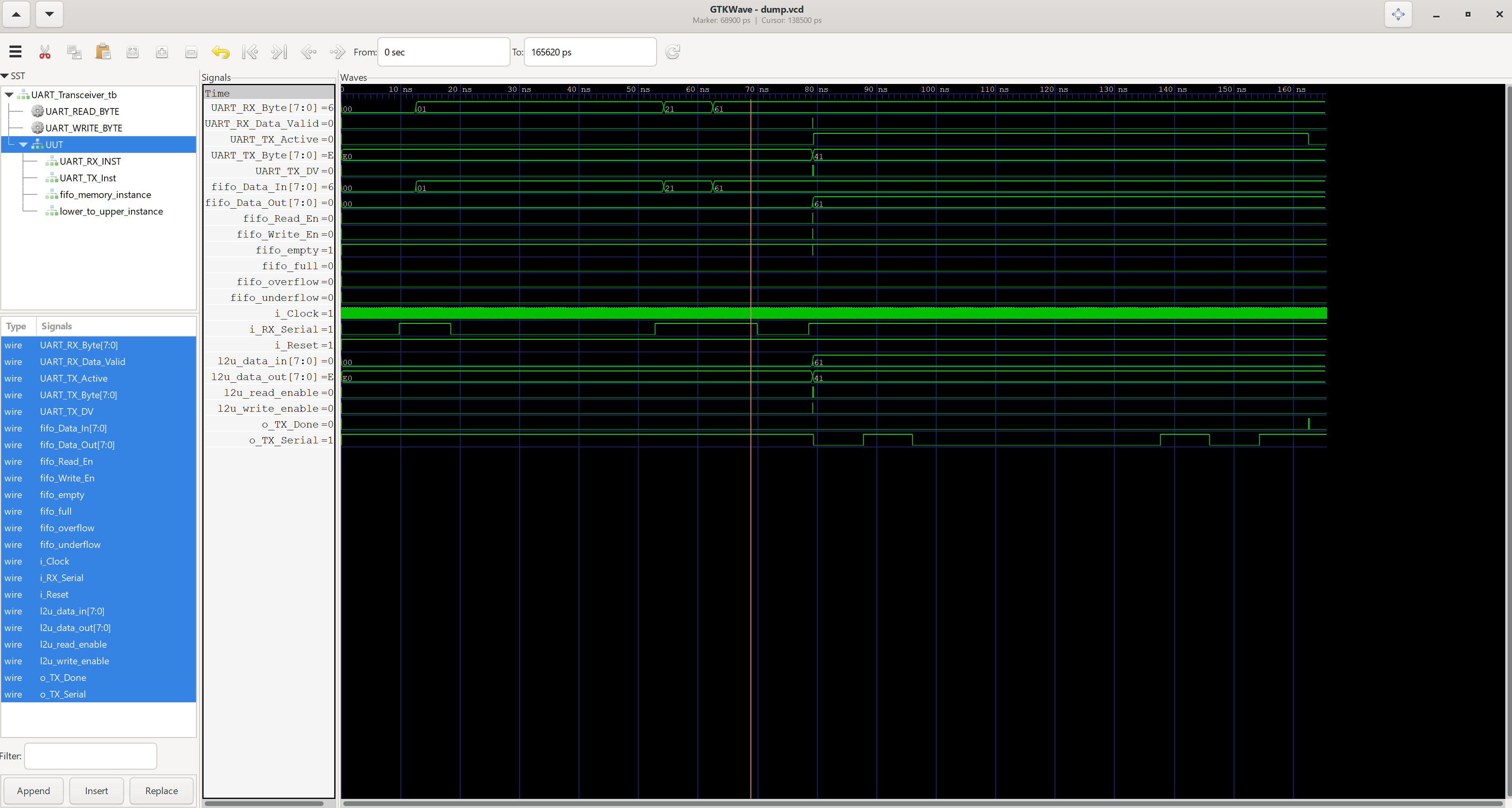Lastly, we can implement this in hardware. We hook up a UART-USB transceiver from our computer to the FPGA board. This is similar to our testbench interaction. You can use any module for that, including the CH340, CP2102, CP2109, FT2232 etc. Use the following `io.adc` file to define your I/O pinout.

``````set_pin_assignment	{ i_Reset }	{ LOCATION = K16; }
set_pin_assignment	{ i_RX_Serial }	{ LOCATION = P2; IOSTANDARD = LVCMOS33; }
set_pin_assignment	{ o_TX_Serial }	{ LOCATION = R2; IOSTANDARD = LVCMOS33; }
set_pin_assignment	{ o_TX_Done }	{ LOCATION = N5; IOSTANDARD = LVCMOS33; }

set_pin_assignment	{ i_Clock }	{ LOCATION = K14; }
``````

Following the steps in Tutorial 1, we set up the environment and generate the bitstream to be uploaded to the board. Below, we can see the resource usage of the FPGA. There’s still plenty of space to do whatever you want on top of this!

``````***Report Model: UART_Transceiver***

IO Statistics
#IO                     5
#input                3
#output               2
#inout                0

Utilization Statistics
#lut                  194   out of  19600    0.99%
#reg                   59   out of  19600    0.30%
#le                   198
#lut only           139   out of    198   70.20%
#reg only             4   out of    198    2.02%
#lut&reg             55   out of    198   27.78%
#dsp                    0   out of     29    0.00%
#bram                   1   out of     64    1.56%
#bram9k               1
#fifo9k               0
#bram32k                0   out of     16    0.00%
#pad                    5   out of    188    2.66%
#ireg                 0
#oreg                 2
#treg                 0
#pll                    0   out of      4    0.00%
``````

Congratulations! You’ve implemented the beginnings of a small computer! For a graphical interface to the FPGA, check out the next tutorial on building a VGA output.

# Tutorial 3: UART Inteface### Tutorial 3: UART Interface

In this tutorial, we will create a UART interface to send and receive data with your computer. This introduces the concept of a state machine to handle incoming data.

Let’s define some parameters for the UART interface we’re using.

• 115200 baud rate
• 8 data bits
• No parity bit
• 1 stop bit
• No flow control

For a detailed explanation of UART, watch this video by nandland. We’ll focus on the implementation in Verilog, and how to use it with the Lichee Tang board.

We use a state machine to perform a sequence of actions to wait for data, look for the start bit, look for data bits, look for the stop bit and clean up the state machine by going back to the `IDLE` state. For our `UART_RX` module, we’ll define a `clk` input and a `RXserial` input, a `datavalid` output and a `RXbyte` bus output.

We’ll also add in the states for our state machine, so we can reference them intuitively instead of relying on values like `3`b001`. We define internal signals as well.

`rClockCount` divides the clock so we only read the data in once, to avoid re-sampling the same data. `rBitIndex` keeps track of which data bit we are currently at, while `rRXByte` keeps track of the actual data. `oRXDataValid`is an output that we use to signal whether the data received is in the correct format, and will be useful for downstream modules that take in data from this UART module. `rSMMain` is the main variable that stores our states for this state machine.

Note that the `case` statement is used for the state machines, rather than daisy-chained `if` `else` blocks.

``````module UART_RX (
input        i_Clock,
input        i_RX_Serial,
output       o_RX_Data_Valid,
output [7:0] o_RX_Byte
);

// States for finite state machine (FSM)
parameter IDLE         = 3'b000;
parameter RX_START_BIT = 3'b001;
parameter RX_DATA_BITS = 3'b010;
parameter RX_STOP_BIT  = 3'b011;
parameter CLEANUP      = 3'b100;

// Internal signals to count clock, keep track of bit position
reg [7:0]     r_Clock_Count   = 0;
reg [2:0]     r_Bit_Index     = 0; //8 bits total
reg [7:0]     r_RX_Byte       = 0;
reg           o_RX_Data_Valid = 0;
reg [2:0]     r_SM_Main       = 0;

endmodule
``````

Now, let’s add in the logic for the state machine itself. The entire state machine is nested within an `always` block, sensitive to `posedge i_Clk`.

The `IDLE` state resets internal signals, and looks for the start bit of `1`b0`. If it’s detected, it goes to the next state.

`RX-START-BIT` uses the internal clock counter to divide the 24MHz clock to 115200, matching the baud rate of the UART line. It uses that to double-check that the start bit has been set, then sets the `RX-DATA-BITS` state. Else, it goes back to the `IDLE` state.

`RX-DATA-BITS` waits `CLKS-PER-BIT -1` clock cycles to sample the incoming data, using the `r-Clock-Count` variable. Once done waiting, in the `else` block, it samples the data bit into the correct position of the `r-RX-Byte[r-Bit-Index]` for storage, until all 8 bits are received. Once the entire byte has been received, it goes to the next state to look for the stop bit.

`RX-STOP-BIT` waits `CLKS-PER-BIT -1` clock cycles, assuming that the stop bit will appear and pass. Then, it sends the state machine to the `CLEANUP` state, and raises high the `o-RX-Data-Valid` signal, to indicate to downstream modules that a data byte is valid, and ready to be read.

`CLEANUP` is the final state, which adds a one clock delay for downstream modules to read the data byte. It then sets the `o-RX-Data-Valid` signal low to indicate that the output data is invalid. Downstream modules can use this signal to ensure they do not read the same output data twice.

Finally, there are some assign statements to tie internal signals to output ports.

``````/////////////////////////////////////////////////////////////////////
/////////////////////////////////////////////////////////////////////
// This file contains the UART Receiver.  This receiver is able to
// receive 8 bits of serial data, one start bit, one stop bit,
// and no parity bit.  When receive is complete o_rx_dv will be
// driven high for one clock cycle.
//
// Set Parameter CLKS_PER_BIT as follows:
// CLKS_PER_BIT = (Frequency of i_Clock)/(Frequency of UART)
// Example: 24 MHz Clock, 115200 baud UART
// (24000000)/(115200) = 208

module UART_RX
#(parameter CLKS_PER_BIT = 208)
(
input        i_Clock,
input  		i_Reset,
input        i_RX_Serial,
output       o_RX_Data_Valid,
output [7:0] o_RX_Byte
);

parameter IDLE         = 3'b000;
parameter RX_START_BIT = 3'b001;
parameter RX_DATA_BITS = 3'b010;
parameter RX_STOP_BIT  = 3'b011;
parameter CLEANUP      = 3'b100;

reg [7:0]     r_Clock_Count   = 0;
reg [2:0]     r_Bit_Index     = 0; //8 bits total
reg [7:0]     r_RX_Byte       = 0;
reg           o_RX_Data_Valid = 0;
reg [2:0]     r_SM_Main       = 0;

// Purpose: Control RX state machine
always @(posedge i_Clock, negedge i_Reset)
begin
if (~i_Reset) begin
o_RX_Data_Valid   <= 1'b0;
r_Clock_Count     <= 0;
r_Bit_Index       <= 0;
r_SM_Main <= IDLE;
end
case (r_SM_Main)
IDLE :
begin
o_RX_Data_Valid   <= 1'b0;
r_Clock_Count     <= 0;
r_Bit_Index       <= 0;

if (i_RX_Serial == 1'b0)          // Start bit detected
r_SM_Main <= RX_START_BIT;
else
r_SM_Main <= IDLE;
end

// Check middle of start bit to make sure it's still low
RX_START_BIT :
begin
if (r_Clock_Count == (CLKS_PER_BIT-1)/2)
begin
if (i_RX_Serial == 1'b0)
begin
r_Clock_Count <= 0;  // reset counter, found the middle
r_SM_Main     <= RX_DATA_BITS;
end
else
r_SM_Main <= IDLE;
end
else
begin
r_Clock_Count <= r_Clock_Count + 1;
r_SM_Main     <= RX_START_BIT;
end
end // case: RX_START_BIT

// Wait CLKS_PER_BIT-1 clock cycles to sample serial data
RX_DATA_BITS :
begin
if (r_Clock_Count < CLKS_PER_BIT-1)
begin
r_Clock_Count <= r_Clock_Count + 1;
r_SM_Main     <= RX_DATA_BITS;
end
else
begin
r_Clock_Count          <= 0;
r_RX_Byte[r_Bit_Index] <= i_RX_Serial;

// Check if we have received all bits
if (r_Bit_Index < 7)
begin
r_Bit_Index <= r_Bit_Index + 1;
r_SM_Main   <= RX_DATA_BITS;
end
else
begin
r_Bit_Index <= 0;
r_SM_Main   <= RX_STOP_BIT;
end
end
end // case: RX_DATA_BITS

// Receive Stop bit.  Stop bit = 1
RX_STOP_BIT :
begin
// Wait CLKS_PER_BIT-1 clock cycles for Stop bit to finish
if (r_Clock_Count < CLKS_PER_BIT-1)
begin
r_Clock_Count <= r_Clock_Count + 1;
r_SM_Main     <= RX_STOP_BIT;
end
else
begin
o_RX_Data_Valid <= 1'b1;
r_Clock_Count   <= 0;
r_SM_Main       <= CLEANUP;
end
end // case: RX_STOP_BIT

// Stay here 1 clock
CLEANUP :
begin
r_SM_Main         <= IDLE;
o_RX_Data_Valid   <= 1'b0;
end

default :
r_SM_Main <= IDLE;

endcase
end

assign o_RX_DV   = o_RX_Data_Valid;
assign o_RX_Byte = r_RX_Byte;

endmodule // UART_RX
``````

Now, let’s write the testbench to ensure that our state machine is able to read and output data correctly.

We start with the `timescale 1ns/10ps`, that defines the timestep to be 1ns, and the minimum resolution to be 10ps. Then, we include our module to be tested `include "UART_RX.v"`.

```````timescale 1ns/10ps
`include "UART_RX.v"

module UART_RX_tb();
endmodule
``````

Then, we define some clocking parameters to simulate the actual clock. These calculations are very rough, but will be good enough to provide behavioural simulation analysis.

```````timescale 1ns/10ps
`include "UART_RX.v"

module UART_RX_tb();

// Testbench uses a 24 MHz clock (same as Lichee Tang board)
// Want to interface to 115200 baud UART
// 24000000 / 115200 = 208 Clocks Per Bit.
parameter c_CLOCK_PERIOD_NS = 41;
parameter c_CLKS_PER_BIT    = 208;
parameter c_BIT_PERIOD      = 8600;

endmodule
``````

Next, we define test signals to send to our top level module.

```````timescale 1ns/10ps
`include "UART_RX.v"

module UART_RX_tb();

// Testbench uses a 24 MHz clock (same as Lichee Tang board)
// Want to interface to 115200 baud UART
// 24000000 / 115200 = 208 Clocks Per Bit.
parameter c_CLOCK_PERIOD_NS = 41;
parameter c_CLKS_PER_BIT    = 208;
parameter c_BIT_PERIOD      = 8600;

reg r_Clock = 0;
reg r_Reset = 1;
reg r_RX_Serial = 1;
wire [7:0] w_RX_Byte;

endomdule
``````

Next, we set up a code block that generates the test signal we want to send to our module. This introduces the `task` and `endtask` keyword. Think of it as a more general function, that takes in multiple inputs and sends out multiple outputs, with specific timing. In this case, it takes in our parallel test data `0x37` and serializes it for the UART receiver test. It sends the start bit, 8 data bits and stop bit with appropriate timings.

``````  // Takes in input byte and serializes it
input [7:0] i_Data;
integer     ii;
begin

// Send Start Bit
r_RX_Serial <= 1'b0;
#(c_BIT_PERIOD);
#1000;

// Send Data Byte
for (ii=0; ii<8; ii=ii+1)
begin
r_RX_Serial <= i_Data[ii];
#(c_BIT_PERIOD);
end

// Send Stop Bit
r_RX_Serial <= 1'b1;
#(c_BIT_PERIOD);
end
``````

Next, let’s initialise our unit under test (UUT), and create our simulation clock.

``````  UART_RX #(.CLKS_PER_BIT(c_CLKS_PER_BIT)) UART_RX_INST
(.i_Clock(r_Clock),
.i_Reset(r_Reset),
.i_RX_Serial(r_RX_Serial),
.o_RX_Data_Valid(),
.o_RX_Byte(w_RX_Byte)
);

always
#(c_CLOCK_PERIOD_NS/2) r_Clock <= !r_Clock;
``````

Lastly, we’ll add in the initial block to pipe signals from our `task` to our UUT, and save all signals to an output waveform for viewing.

``````  // Main Testing:
initial
begin
// Send a command to the UART (exercise Rx)
@(posedge r_Clock);
UART_WRITE_BYTE(8'h37);
@(posedge r_Clock);

// Check that the correct command was received
if (w_RX_Byte == 8'h37)
\$display("Test Passed - Correct Byte Received");
else
\$display("Test Failed - Incorrect Byte Received");
\$finish();
end

initial
begin
// Required to dump signals to EPWave
\$dumpfile("dump.vcd");
\$dumpvars(0);
end
``````

Bringing it all together, here’s the final `testbench` Verilog file.

``````//////////////////////////////////////////////////////////////////////
//////////////////////////////////////////////////////////////////////

// This testbench will exercise the UART RX.
// It sends out byte 0x37, and ensures the RX receives it correctly.
`timescale 1ns/10ps
`include "UART_RX.v"

module UART_RX_tb();

// Testbench uses a 24 MHz clock (same as Lichee Tang board)
// Want to interface to 115200 baud UART
// 24000000 / 115200 = 208 Clocks Per Bit.
parameter c_CLOCK_PERIOD_NS = 41;
parameter c_CLKS_PER_BIT    = 208;
parameter c_BIT_PERIOD      = 8600;

reg r_Clock = 0;
reg r_Reset = 1;
reg r_RX_Serial = 1;
wire [7:0] w_RX_Byte;

// Takes in input byte and serializes it
input [7:0] i_Data;
integer     ii;
begin

// Send Start Bit
r_RX_Serial <= 1'b0;
#(c_BIT_PERIOD);
#1000;

// Send Data Byte
for (ii=0; ii<8; ii=ii+1)
begin
r_RX_Serial <= i_Data[ii];
#(c_BIT_PERIOD);
end

// Send Stop Bit
r_RX_Serial <= 1'b1;
#(c_BIT_PERIOD);
end

UART_RX #(.CLKS_PER_BIT(c_CLKS_PER_BIT)) UART_RX_INST
(.i_Clock(r_Clock),
.i_Reset(r_Reset),
.i_RX_Serial(r_RX_Serial),
.o_RX_Data_Valid(),
.o_RX_Byte(w_RX_Byte)
);

always
#(c_CLOCK_PERIOD_NS/2) r_Clock <= !r_Clock;

// Main Testing:
initial
begin
// Send a command to the UART (exercise Rx)
@(posedge r_Clock);
UART_WRITE_BYTE(8'h37);
@(posedge r_Clock);

// Check that the correct command was received
if (w_RX_Byte == 8'h37)
\$display("Test Passed - Correct Byte Received");
else
\$display("Test Failed - Incorrect Byte Received");
\$finish();
end

initial
begin
// Required to dump signals
\$dumpfile("dump.vcd");
\$dumpvars(0);
end

endmodule
``````

Congratulations! We’ve successfully followed nandland’s tutorial on building a UART receiver. Now, let’s move on to building the UART transmitter.

#### UART Transmitter

For the UART transmitter, it’s quite similar to the `task` from the UART receiver testbench. The variable names are also similar to the UART receiver module. We define an additional signal, `r-TX-Active` to indicate when the transmitter is active. This allows you to handle half-duplex applications where you transmit and receive on the same line.

``````module UART_TX
#(parameter CLKS_PER_BIT = 208)
(
input       i_Clock,
input       i_Reset,
input       i_TX_DV,
input [7:0] i_TX_Byte,
output      o_TX_Active,
output reg  o_TX_Serial,
output      o_TX_Done
);

parameter IDLE         = 3'b000;
parameter TX_START_BIT = 3'b001;
parameter TX_DATA_BITS = 3'b010;
parameter TX_STOP_BIT  = 3'b011;
parameter CLEANUP      = 3'b100;

reg [2:0] r_SM_Main     = 0;
reg [7:0] r_Clock_Count = 0;
reg [2:0] r_Bit_Index   = 0;
reg [7:0] r_TX_Data     = 0;
reg       r_TX_Done     = 0;
reg       r_TX_Active   = 0;

endmodule
``````

Next, we define the behaviour of the module. Within the main `always` block of the code, we have the state machine for the UART transmitter.

`IDLE` initialises the values of the output and internal signals, waiting for a ready signal on `i-TX-DV`. Once available, it saves the data to be sent `i-TX-Data` and goes to the next state.

`TX-START-BIT` sends out the start bit, then waits for it to finish to adhere to timing. Once done, it moves to the next state to start sending data bits.

`TX-DATA-BITS` handles the sending of the data, one bit at a time, according to the `r-Bit-Index` variable. It adheres to timing by waiting the appropriate amount of time after setting each output bit, effectively converting from parallel to serial data.

`TX-STOP-BIT` state sets the stop bit, waits for some time, then sets the `r-TX-Done` flag to signal that transmission of the byte has finished. It then resets some internal signals, before moving on to the next state.

`CLEANUP` state waits for one clock cycle, before going back to the `IDLE` state to wait for more data to be sent. Lastly, some `assign` statements connect internal signals to output ports.

``````  always @(posedge i_Clock, negedge i_Reset)
begin
if (!i_Reset) begin
r_SM_Main <= IDLE;
end
case (r_SM_Main)
IDLE :
begin
o_TX_Serial   <= 1'b1;         // Drive Line High for Idle
r_TX_Done     <= 1'b0;
r_Clock_Count <= 0;
r_Bit_Index   <= 0;

if (i_TX_DV == 1'b1)
begin
r_TX_Active <= 1'b1;
r_TX_Data   <= i_TX_Byte;
r_SM_Main   <= TX_START_BIT;
end
else
r_SM_Main <= IDLE;
end // case: IDLE

// Send out Start Bit. Start bit = 0
TX_START_BIT :
begin
o_TX_Serial <= 1'b0;

// Wait CLKS_PER_BIT-1 clock cycles for start bit to finish
if (r_Clock_Count < CLKS_PER_BIT-1)
begin
r_Clock_Count <= r_Clock_Count + 1;
r_SM_Main     <= TX_START_BIT;
end
else
begin
r_Clock_Count <= 0;
r_SM_Main     <= TX_DATA_BITS;
end
end // case: TX_START_BIT

// Wait CLKS_PER_BIT-1 clock cycles for data bits to finish
TX_DATA_BITS :
begin
o_TX_Serial <= r_TX_Data[r_Bit_Index];

if (r_Clock_Count < CLKS_PER_BIT-1)
begin
r_Clock_Count <= r_Clock_Count + 1;
r_SM_Main     <= TX_DATA_BITS;
end
else
begin
r_Clock_Count <= 0;

// Check if we have sent out all bits
if (r_Bit_Index < 7)
begin
r_Bit_Index <= r_Bit_Index + 1;
r_SM_Main   <= TX_DATA_BITS;
end
else
begin
r_Bit_Index <= 0;
r_SM_Main   <= TX_STOP_BIT;
end
end
end // case: TX_DATA_BITS

// Send out Stop bit.  Stop bit = 1
TX_STOP_BIT :
begin
o_TX_Serial <= 1'b1;

// Wait CLKS_PER_BIT-1 clock cycles for Stop bit to finish
if (r_Clock_Count < CLKS_PER_BIT-1)
begin
r_Clock_Count <= r_Clock_Count + 1;
r_SM_Main     <= TX_STOP_BIT;
end
else
begin
r_TX_Done     <= 1'b1;
r_Clock_Count <= 0;
r_SM_Main     <= CLEANUP;
r_TX_Active   <= 1'b0;
end
end // case: TX_STOP_BIT

// Stay here 1 clock
CLEANUP :
begin
r_TX_Done <= 1'b1;
r_SM_Main <= IDLE;
end

default :
r_SM_Main <= IDLE;

endcase
end

assign o_TX_Active = r_TX_Active;
assign o_TX_Done   = r_TX_Done;
``````

s

``````//////////////////////////////////////////////////////////////////////
//////////////////////////////////////////////////////////////////////
// This file contains the UART Transmitter.  This transmitter is able
// to transmit 8 bits of serial data, one start bit, one stop bit,
// and no parity bit.  When transmit is complete o_Tx_done will be
// driven high for one clock cycle.
//
// Set Parameter CLKS_PER_BIT as follows:
// CLKS_PER_BIT = (Frequency of i_Clock)/(Frequency of UART)
// Example: 24 MHz Clock, 115200 baud UART
// (24000000)/(115200) = 208

module UART_TX
#(parameter CLKS_PER_BIT = 208)
(
input       i_Clock,
input       i_Reset,
input       i_TX_DV,
input [7:0] i_TX_Byte,
output      o_TX_Active,
output reg  o_TX_Serial,
output      o_TX_Done
);

parameter IDLE         = 3'b000;
parameter TX_START_BIT = 3'b001;
parameter TX_DATA_BITS = 3'b010;
parameter TX_STOP_BIT  = 3'b011;
parameter CLEANUP      = 3'b100;

reg [2:0] r_SM_Main     = 0;
reg [7:0] r_Clock_Count = 0;
reg [2:0] r_Bit_Index   = 0;
reg [7:0] r_TX_Data     = 0;
reg       r_TX_Done     = 0;
reg       r_TX_Active   = 0;

always @(posedge i_Clock, negedge i_Reset)
begin
if (!i_Reset) begin
r_SM_Main <= IDLE;
end
case (r_SM_Main)
IDLE :
begin
o_TX_Serial   <= 1'b1;         // Drive Line High for Idle
r_TX_Done     <= 1'b0;
r_Clock_Count <= 0;
r_Bit_Index   <= 0;

if (i_TX_DV == 1'b1)
begin
r_TX_Active <= 1'b1;
r_TX_Data   <= i_TX_Byte;
r_SM_Main   <= TX_START_BIT;
end
else
r_SM_Main <= IDLE;
end // case: IDLE

// Send out Start Bit. Start bit = 0
TX_START_BIT :
begin
o_TX_Serial <= 1'b0;

// Wait CLKS_PER_BIT-1 clock cycles for start bit to finish
if (r_Clock_Count < CLKS_PER_BIT-1)
begin
r_Clock_Count <= r_Clock_Count + 1;
r_SM_Main     <= TX_START_BIT;
end
else
begin
r_Clock_Count <= 0;
r_SM_Main     <= TX_DATA_BITS;
end
end // case: TX_START_BIT

// Wait CLKS_PER_BIT-1 clock cycles for data bits to finish
TX_DATA_BITS :
begin
o_TX_Serial <= r_TX_Data[r_Bit_Index];

if (r_Clock_Count < CLKS_PER_BIT-1)
begin
r_Clock_Count <= r_Clock_Count + 1;
r_SM_Main     <= TX_DATA_BITS;
end
else
begin
r_Clock_Count <= 0;

// Check if we have sent out all bits
if (r_Bit_Index < 7)
begin
r_Bit_Index <= r_Bit_Index + 1;
r_SM_Main   <= TX_DATA_BITS;
end
else
begin
r_Bit_Index <= 0;
r_SM_Main   <= TX_STOP_BIT;
end
end
end // case: TX_DATA_BITS

// Send out Stop bit.  Stop bit = 1
TX_STOP_BIT :
begin
o_TX_Serial <= 1'b1;

// Wait CLKS_PER_BIT-1 clock cycles for Stop bit to finish
if (r_Clock_Count < CLKS_PER_BIT-1)
begin
r_Clock_Count <= r_Clock_Count + 1;
r_SM_Main     <= TX_STOP_BIT;
end
else
begin
r_TX_Done     <= 1'b1;
r_Clock_Count <= 0;
r_SM_Main     <= CLEANUP;
r_TX_Active   <= 1'b0;
end
end // case: TX_STOP_BIT

// Stay here 1 clock
CLEANUP :
begin
r_TX_Done <= 1'b1;
r_SM_Main <= IDLE;
end

default :
r_SM_Main <= IDLE;

endcase
end

assign o_TX_Active = r_TX_Active;
assign o_TX_Done   = r_TX_Done;

endmodule
``````

Next, let’s set up a testbench to simulate the UART transmitter and receiver in loopback mode, where the transmitter connects to the receiver.

``````//////////////////////////////////////////////////////////////////////
//////////////////////////////////////////////////////////////////////

// This testbench will exercise the UART RX.
// It sends out byte 0x37, and ensures the RX receives it correctly.
`timescale 1ns/10ps

`include "UART_TX.v"

module UART_TX_TB ();

// Testbench uses a 24 MHz clock (same as Lichee Tang board)
// Want to interface to 115200 baud UART
// 24000000 / 115200 = 208 Clocks Per Bit.
parameter c_CLOCK_PERIOD_NS = 41;
parameter c_CLKS_PER_BIT    = 208;
parameter c_BIT_PERIOD      = 8600;

reg r_Clock = 0;
reg r_Reset = 1;
reg r_TX_DV = 0;
wire w_TX_Active, w_UART_Line;
wire w_TX_Serial;
reg [7:0] r_TX_Byte = 0;
wire [7:0] w_RX_Byte;

UART_RX #(.CLKS_PER_BIT(c_CLKS_PER_BIT)) UART_RX_Inst
(.i_Clock(r_Clock),
.i_Reset(r_Reset),
.i_RX_Serial(w_UART_Line),
.o_RX_DV(w_RX_DV),
.o_RX_Byte(w_RX_Byte)
);

UART_TX #(.CLKS_PER_BIT(c_CLKS_PER_BIT)) UART_TX_Inst
(.i_Clock(r_Clock),
.i_Reset(r_Reset),
.i_TX_DV(r_TX_DV),
.i_TX_Byte(r_TX_Byte),
.o_TX_Active(w_TX_Active),
.o_TX_Serial(w_TX_Serial),
.o_TX_Done()
);

// Keeps the UART Receive input high (default) when
// UART transmitter is not active
assign w_UART_Line = w_TX_Active ? w_TX_Serial : 1'b1;

always
#(c_CLOCK_PERIOD_NS/2) r_Clock <= !r_Clock;

// Main Testing:
initial
begin
// Tell UART to send a command (exercise TX)
@(posedge r_Clock);
@(posedge r_Clock);
r_TX_DV   <= 1'b1;
r_TX_Byte <= 8'h3F;
@(posedge r_Clock);
r_TX_DV <= 1'b0;

// Check that the correct command was received
@(posedge w_RX_DV);
if (w_RX_Byte == 8'h3F)
\$display("Test Passed - Correct Byte Received");
else
\$display("Test Failed - Incorrect Byte Received");
\$finish();
end

initial
begin
// Required to dump signals to EPWave
\$dumpfile("dump.vcd");
\$dumpvars(0);
end
endmodule
``````

Finally, we’ve implemented a basic UART peripheral! You’ll note that this only allows you to receive one byte at a time, and has no buffer to store the last few bytes. This means that whatever you send will push old data out of the system, which usually isn’t desirable when you don’t know when the next data byte will come - fast or slow.

# Tutorial 2: Seven segment display### Tutorial 2: Seven Segment Display

In this tutorial, we will control a seven segment display using the FPGA. This will introduce concepts such as `module` instantiation where code can be written and reused, a similar paradigm to Object Oriented Programming.

A seven segment display is basically a package of seven/eight LEDs that allow you to form numbers 0-f by lighting them up in specific formats. In this tutorial, we use a common anode version, where the anodes are connected, and pulled `HIGH`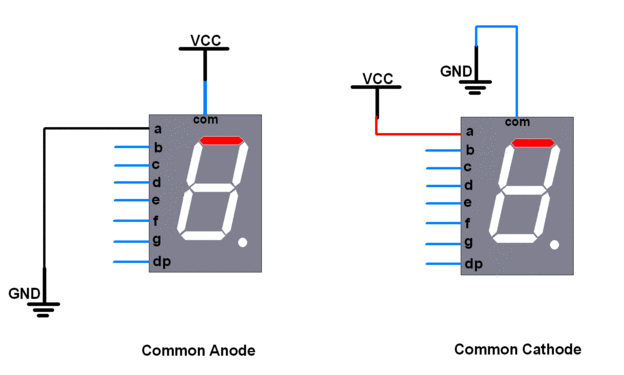We start by looking at which combinations of LEDs to light up, to show a specific digit on the display. The truth table for the LED inputs for a given output digit is shown below.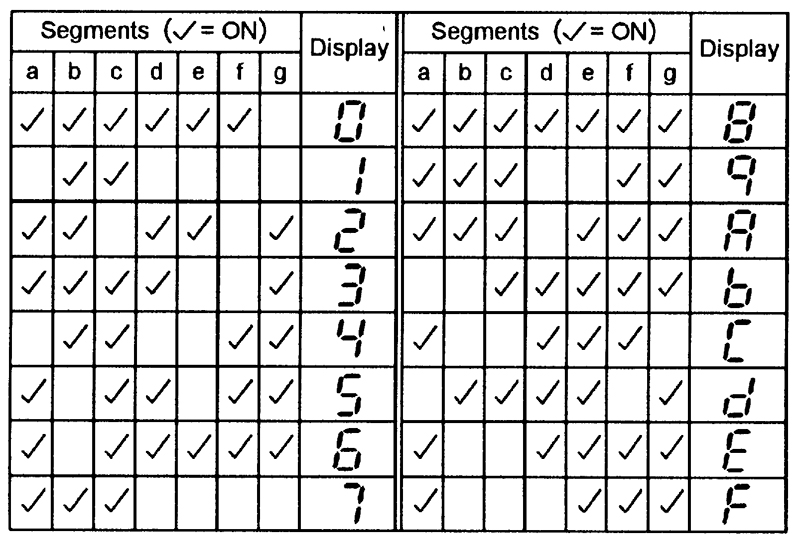Let’s create a module to light up the LEDs in the correct combination. The module takes a 4-bit input (0 to F in hex) and lights up the corresponding digit. We start by including the truth table as a set of parameters that we can call during operation.

``````module Seven_Segment
(
input wire CLK_IN,
input wire [3:0] NUMBER_IN,
output reg [6:0] OUTPUT
);

parameter zero   = 7'b1111110;  //Value for zero
parameter one    = 7'b0110000;  //Value for one
parameter two    = 7'b1101101;  //Value for two
parameter three  = 7'b1111001;  //Value for three
parameter four   = 7'b0110011;  //Value for four
parameter five   = 7'b1011011;  //Value for five
parameter six    = 7'b1011111;  //Value for six
parameter seven  = 7'b1110000;  //Value for seven
parameter eight  = 7'b1111111;  //Value for eight
parameter nine   = 7'b1110011;  //Value for nine
parameter A      = 7'b1110111;  //Value for A
parameter B      = 7'b0011111;  //Value for B
parameter C      = 7'b1001110;  //Value for C
parameter D      = 7'b0111101;  //Value for D
parameter E      = 7'b1001111;  //Value for E
parameter F      = 7'b1000111;  //Value for F

endmodule
``````

Then, we’ll want to define the behaviour of the module at each clock pulse with an `always` block. We synchronise this module to a clock’s rising edge `posedge`, so that we can update the value on the display whenever we get a new input. You can also use the falling edge with `negedge`. Most modules in FPGAs will be synchronised to a clock, allowing you to pipeline data from one module to another sequentially. This is a very important concept in FPGA design, as you will see in more advanced tutorials.

``````module Seven_Segment
(
input wire CLK_IN,
input wire [3:0] NUMBER_IN,
output reg [6:0] OUTPUT
);

parameter zero   = 7'b1111110;  //Value for zero
parameter one    = 7'b0110000;  //Value for one
parameter two    = 7'b1101101;  //Value for two
parameter three  = 7'b1111001;  //Value for three
parameter four   = 7'b0110011;  //Value for four
parameter five   = 7'b1011011;  //Value for five
parameter six    = 7'b1011111;  //Value for six
parameter seven  = 7'b1110000;  //Value for seven
parameter eight  = 7'b1111111;  //Value for eight
parameter nine   = 7'b1110011;  //Value for nine
parameter A      = 7'b1110111;  //Value for A
parameter B      = 7'b0011111;  //Value for B
parameter C      = 7'b1001110;  //Value for C
parameter D      = 7'b0111101;  //Value for D
parameter E      = 7'b1001111;  //Value for E
parameter F      = 7'b1000111;  //Value for F

always @(posedge CLK_IN) begin
// Do something
end
endmodule
``````

Inside the `always` block, we define the behaviour of the outputs. We invert the output with a `~` operator, as we are using a common anode display. We need to drive the selected LED a/b/c/d/e/f/g `LOW` to turn it on. Save this file as `Seven_Segment.v`.

``````module Seven_Segment (
input wire CLK_IN,
input wire [3:0]NUMBER_IN,
output reg [6:0] OUTPUT
);

parameter zero   = 7'b1111110;  //Value for zero
parameter one    = 7'b0110000;  //Value for one
parameter two    = 7'b1101101;  //Value for two
parameter three  = 7'b1111001;  //Value for three
parameter four   = 7'b0110011;  //Value for four
parameter five   = 7'b1011011;  //Value for five
parameter six    = 7'b1011111;  //Value for six
parameter seven  = 7'b1110000;  //Value for seven
parameter eight  = 7'b1111111;  //Value for eight
parameter nine   = 7'b1110011;  //Value for nine
parameter A      = 7'b1110111;  //Value for A
parameter B      = 7'b0011111;  //Value for B
parameter C      = 7'b1001110;  //Value for C
parameter D      = 7'b0111101;  //Value for D
parameter E      = 7'b1001111;  //Value for E
parameter F      = 7'b1000111;  //Value for F

always @(posedge CLK_IN) begin
case(NUMBER_IN)
4'b0000: OUTPUT <= ~zero;
4'b0001: OUTPUT <= ~one;
4'b0010: OUTPUT <= ~two;
4'b0011: OUTPUT <= ~three;
4'b0100: OUTPUT <= ~four;
4'b0101: OUTPUT <= ~five;
4'b0110: OUTPUT <= ~six;
4'b0111: OUTPUT <= ~seven;
4'b1000: OUTPUT <= ~eight;
4'b1001: OUTPUT <= ~nine;
4'b1010: OUTPUT <= ~A;
4'b1011: OUTPUT <= ~B;
4'b1100: OUTPUT <= ~C;
4'b1101: OUTPUT <= ~D;
4'b1110: OUTPUT <= ~E;
4'b1111: OUTPUT <= ~F;
default: OUTPUT <= ~zero;
endcase
end
endmodule
``````

Now, we’ve created a module that takes in a 4-bit input and displays the corresponding digit on the seven segment display. Let’s do something more advanced. Now, we have a 4-digit seven segment display, as shown below. Let’s show a 16-bit number on it!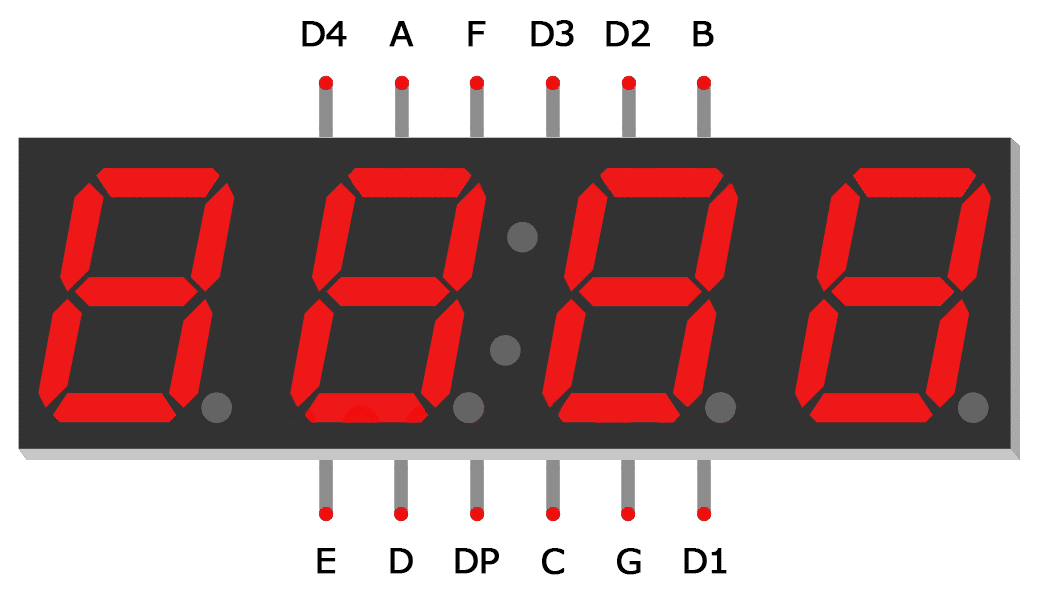Now we have some additional pins, D1-D4. These are used to select the corresponding digit in the display, by driving it `HIGH` and the segment side `LOW` to create a voltage difference across the LED segment, lighting it up.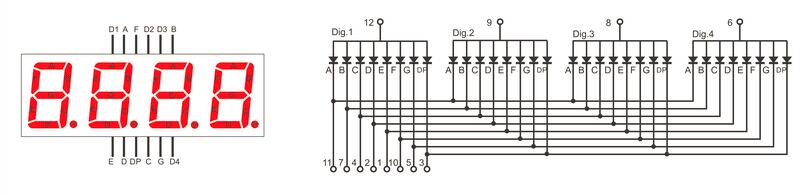How do you light up so many digits if they share a common pin? The answer is simple: LED multiplexing! What you need to do is continuously switch on and off the correct digit so fast that it appears as one continuous image to the naked eye. For that, you’ll need a refresh rate of at least 60Hz. We can comfortably achieve that and a lot more with our mighty FPGA.

In our module, we’ll define a 16-bit input, representing the number we want to display. Our outputs will be all the pins of this 4-digit seven segment display. First, we start by including our `Seven_Segment.v` module with `include`.

```````include "Seven_Segment.v"

module Seven_Segment_Display (
input wire clk,
input wire RST_N,
input wire [15:0] Displayed_number,
output reg [3:0] Cathode,
output wire [6:0] Segment_out
);

endmodule
``````

We instantiate our `Seven_Segment.v` module as shown below, adding a signal `Digitnumber` to send the 4-bit digit to the module.

```````include "Seven_Segment.v"

module Seven_Segment_Display (
input wire clk,
input wire RST_N,
input wire [15:0] Displayed_number,
output reg [3:0] Cathode,
output wire [6:0] Segment_out
);

reg [3:0] Digit_number;

// Creating Seven_Segment instance
Seven_Segment i2
(
.CLK_IN(clk),
.NUMBER_IN(Digit_number),
.OUTPUT(Segment_out[6:0])
);

endmodule
``````

Then, we add in our logic to alternate between the 4 digits of the seven segment display, to rapidly display all digits on them. We use a 2-bit counter `LEDactivatingcounter` to choose which one to light up, and `DigitNumber` to represent the 4-bit digit displayed on the current display.

```````include "Seven_Segment.v"

module Seven_Segment_Display (
input wire clk,
input wire RST_N,
input wire [15:0] Displayed_number,
output reg [3:0] Cathode,
output wire [6:0] Segment_out
);

wire [1:0] LED_activating_counter;
reg [3:0] Digit_number;
reg [15:0] refresh_counter;

// Creating Seven_Segment instance
Seven_Segment i2
(
.CLK_IN(clk),
.NUMBER_IN(Digit_number),
.OUTPUT(Segment_out[6:0])
);

// Switch between 4 digits of display
always @(posedge clk or negedge RST_N)
begin
if (RST_N==0)
refresh_counter <= 0;
else
refresh_counter <= refresh_counter + 1;
end

// every 24M / (2^14) hz switch to next digit in 7-seg display
assign LED_activating_counter = refresh_counter[15:14];

// select digit to light up
always @(posedge clk) begin
case(LED_activating_counter)
2'b00: begin
// pull to ground for first digit
Cathode = 4'b1000;
Digit_number <= Displayed_number[15:11];
end
2'b01: begin
// pull to ground for second digit
Cathode = 4'b0100;
Digit_number <= Displayed_number[10:8];
end
2'b10: begin
// pull to ground for third digit
Cathode = 4'b0010;
Digit_number <= Displayed_number[7:4];
end
2'b11: begin
// pull to ground for fourth digit
Cathode = 4'b0001;
Digit_number <= Displayed_number[3:0];
end
default: begin
// pull to ground for default first digit
Cathode <= 4'b1111;
Digit_number <= 4'b1111;
end
endcase
end

endmodule
``````

Let’s take a closer look at the code above. For our `always` block sensitivity list, we added the reset signal `negedge rst` to incorporate our reset button, which is active low.

We see a new construct here, the `case` block. Similar to C, the case statement checks the input value and behaves accordingly. In this case, we check for values 0-3 to light up digits 1-4 respectively. This block is nested within an `always` block to synchronise it with the master clock. This module is enough to display a 16-bit number on the 4-digit seven segment display.

Now, let’s add a Fibonacci counter to automatically increment the number displayed. This module increments the output `SEQUENCE` at every clock cycle by adding the previous two values together. Note that when this value overflows, it resets back to 0. Save this file as `Fibonacci_Series.v`.

``````module Fibonacci_Series (
input wire CLK_IN,
input wire RST_N,
output wire [15:0]SEQUENCE
);

reg [15:0] SEQUENCE_I1,SEQUENCE_I2;

assign SEQUENCE = RST_N ? (SEQUENCE_I1 + SEQUENCE_I2) : 16'b1;

always @(posedge CLK_IN) begin
if(SEQUENCE < 16'hDAAA) begin
SEQUENCE_I2 = SEQUENCE_I1;
SEQUENCE_I1 = SEQUENCE;
end
else begin
SEQUENCE_I2 = 16'b1;
SEQUENCE_I1 = 16'b0;
end
end
endmodule
``````

The Lichee Tang has an onboard 24MHz clock that we take in on pin `K14`. We divide that clock to get a slower clock to trigger the `Fibonacci_Series` module, incrementing it slowly.

```````include "Seven_Segment_Display.v"
`include "Fibonacci_Series.v"

module Seven_Segment_Display_Top (
input wire clk,
input wire RST_N,
output wire [3:0] Cathode,
output wire [6:0] Segment_out
);

// Signal to send number to Seven_Segment_Display module
wire [15:0] Displayed_number;

// Frequency of master clock
parameter time1 = 25'd24_000_000;  // 24 MHz counter

// Slow clock divider
reg [24:0] count = 24'b0;
reg clk_slow = 1'b0;

// Slow clock to increment number displayed
always @(posedge clk) begin
// Code for reset
if(RST_N==0) begin
count <= 25'd0;
clk_slow <= 1'b0;
end
if(count == time1) begin
count <= 25'd0;
clk_slow <= ~clk_slow;
end
else begin
count <= count + 1'b1;
end
end

// Creating Fibonacci_Series instance
Fibonacci_Series i1
(
.CLK_IN(clk_slow),
.RST_N(RST_N),
.SEQUENCE(Displayed_number[15:0])
);

// Creating 4-digit seven segment display instance
Seven_Segment_Display Seven_Segment_Display_inst
(
.clk(clk),
.RST_N(RST_N),
.Displayed_number(Displayed_number),
.Cathode(Cathode),
.Segment_out(Segment_out)
);

endmodule
``````

Save our top module. Now, let’s create a testbench to simulate our top module, ensuring that the output signals are as expected.

```````timescale 1ns/1ns
`include "Seven_Segment_Display_Top.v"

module Seven_Segment_Display_Top_tb ();

// Test signals
reg clk = 1'b0;
reg RST_N = 1'b1;
wire [3:0] Cathode;
wire [6:0] Segment_out;

// Instantiate the top module
Seven_Segment_Display_Top uut
(
.clk(clk),
.RST_N(RST_N),
.Cathode(Cathode),
.Segment_out(Segment_out)
);

initial begin
// Define testbench behaviour
\$dumpfile("Seven_Segment_Display_Top_tb.vcd");
\$dumpvars(0, Seven_Segment_Display_Top_tb);

// Test conditions
for (integer i=0; i<10; i=i+1) begin
// Pulse clock, 20 units per cycle
clk = ~clk; #10;
end
\$display("Test completed!");
end

endmodule
``````

Before running the simulation, let’s make some small changes to allow the simulation to take effect in a small number of timesteps. The simulation only does the 10~1000s of steps, whereas your hardware implementation will do 24,000,000 in a single second at 24MHz. I’ve added a parameter to the children modules (accessible from the top module) to define a faster “slow clock”, so we can see changes in fewer timesteps.

`SevenSegmentDisplay.v`

```````include "Seven_Segment.v"

module Seven_Segment_Display (
input wire clk,
input wire RST_N,
input wire [15:0] Displayed_number,
output reg [3:0] Cathode,
output wire [6:0] Segment_out
);

// For modification during simulation later
parameter startRefreshCounter = 14;
parameter endRefreshCounter = 15;

wire [1:0] LED_activating_counter;
reg [3:0] Digit_number;
reg [15:0] refresh_counter = 16'b0;

// Creating Seven_Segment instance
Seven_Segment i2
(
.CLK_IN(clk),
.NUMBER_IN(Digit_number),
.OUTPUT(Segment_out[6:0])
);

// Switch between 4 digits of display
always @(posedge clk or negedge RST_N)
begin
if (RST_N==0)
refresh_counter <= 0;
else
refresh_counter <= refresh_counter + 1;
end

// every 24M / (2^14) hz switch to next digit in 7-seg display
assign LED_activating_counter = refresh_counter[endRefreshCounter:startRefreshCounter];

// select digit to light up
always @(posedge clk) begin
case(LED_activating_counter)
2'b00: begin
// pull to ground for first digit
Cathode = 4'b1000;
Digit_number <= Displayed_number[15:11];
end
2'b01: begin
// pull to ground for second digit
Cathode = 4'b0100;
Digit_number <= Displayed_number[10:8];
end
2'b10: begin
// pull to ground for third digit
Cathode = 4'b0010;
Digit_number <= Displayed_number[7:4];
end
2'b11: begin
// pull to ground for fourth digit
Cathode = 4'b0001;
Digit_number <= Displayed_number[3:0];
end
default: begin
// pull to ground for default first digit
Cathode <= 4'b1111;
Digit_number <= 4'b1111;
end
endcase
end

endmodule
``````

`SevenSegmentDisplayTop.v`

```````include "Seven_Segment_Display.v"
`include "Fibonacci_Series.v"

module Seven_Segment_Display_Top (
input wire clk,
input wire RST_N,
output wire [3:0] Cathode,
output wire [6:0] Segment_out
);

// Signal to send number to Seven_Segment_Display module
wire [15:0] Displayed_number;

// For modification during simulation later
parameter startRefreshCounter = 14;
parameter endRefreshCounter = 15;

// Frequency of master clock
parameter time1 = 25'd24_000_000;  // 24 MHz counter

// Slow clock divider
reg [24:0] count = 24'b0;
reg clk_slow = 1'b0;

// Slow clock to increment number displayed
always @(posedge clk) begin
// Code for reset
if(RST_N==0) begin
count <= 25'd0;
clk_slow <= 1'b0;
end
if(count == time1) begin
count <= 25'd0;
clk_slow <= ~clk_slow;
end
else begin
count <= count + 1'b1;
end
end

// Creating Fibonacci_Series instance
Fibonacci_Series i1
(
.CLK_IN(clk_slow),
.RST_N(RST_N),
.SEQUENCE(Displayed_number)
);

// Creating 4-digit seven segment display instance
Seven_Segment_Display #(
.startRefreshCounter(startRefreshCounter),
.endRefreshCounter(endRefreshCounter)
) Seven_Segment_Display_inst
(
.clk(clk),
.RST_N(RST_N),
.Displayed_number(Displayed_number),
.Cathode(Cathode),
.Segment_out(Segment_out)
);

endmodule
``````

`testbench`

```````timescale 1ns/1ns
`include "Seven_Segment_Display_Top.v"

module Seven_Segment_Display_Top_tb ();

// Test signals
reg clk = 1'b0;
reg RST_N = 1'b1;
wire [3:0] Cathode;
wire [6:0] Segment_out;

// Instantiate the top module
Seven_Segment_Display_Top #(
// Change parameters for simulation purposes, to speed up changes
.time1(2),
.startRefreshCounter(0),
.endRefreshCounter(1)
) uut
(
.clk(clk),
.RST_N(RST_N),
.Cathode(Cathode),
.Segment_out(Segment_out)
);

initial begin
// Define testbench behaviour
\$dumpfile("Seven_Segment_Display_Top_tb.vcd");
\$dumpvars(0, Seven_Segment_Display_Top_tb);

// Test conditions
for (integer i=0; i<100; i=i+1) begin
// Pulse clock, 20 units per cycle
clk = ~clk; #10;
end
\$display("Test completed!");
end

endmodule
``````

Run the file with the following commands.

``````iverilog -o Seven_Segment_Display_Top_tb.vvp Seven_Segment_Display_Top_tb.v

vvp Seven_Segment_Display_Top_tb.vvp

gtkwave Seven_Segment_Display_Top_tb.vcd
``````

We get the following output waveform when viewed in `gtkwave`.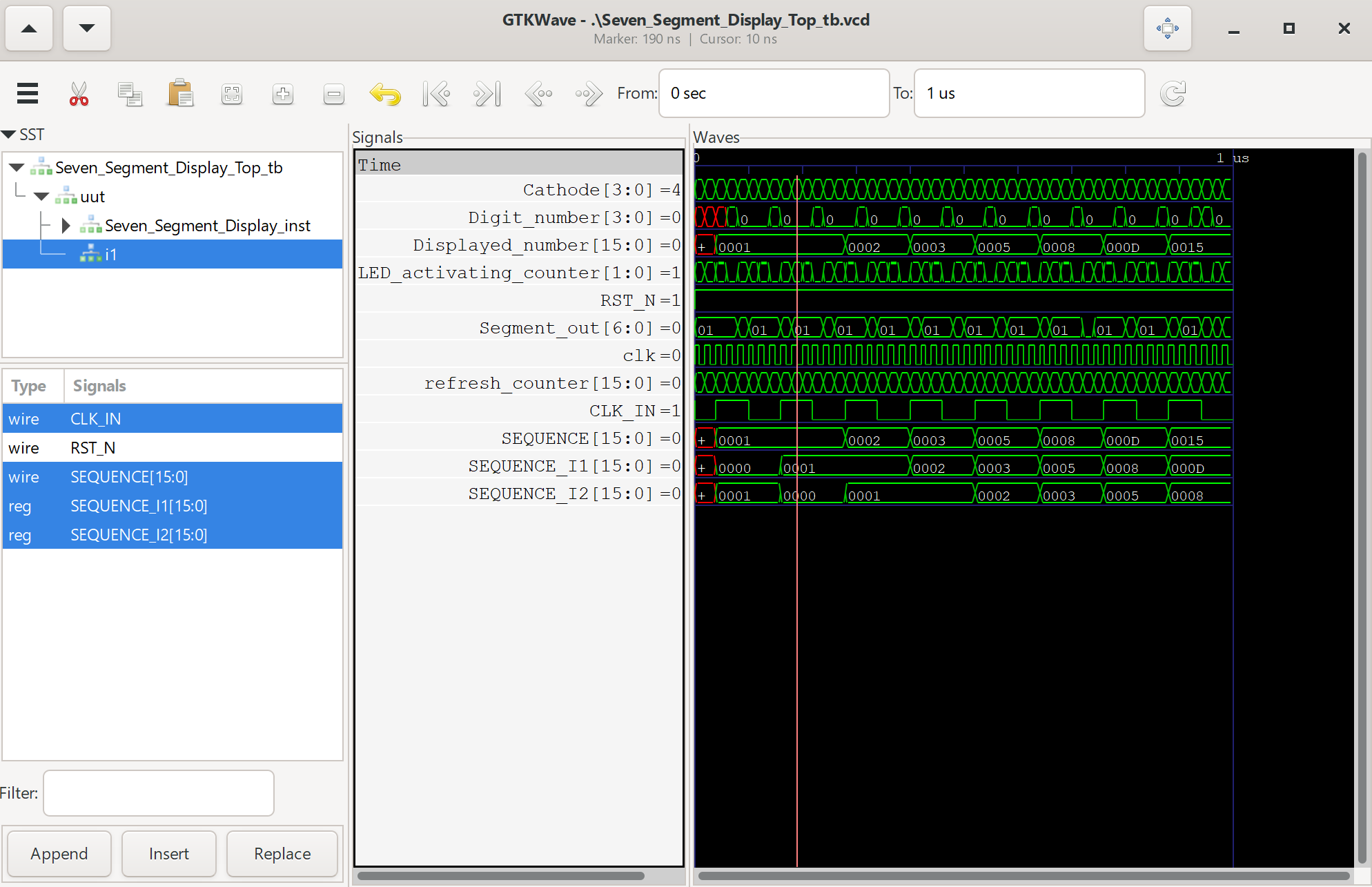Now, we’ve finally finished this seven segment display project that displays Fibonacci numbers up to the 16-bit limit of `FFFF` in hexadecimal. Let’s try deploying it to hardware with the following steps:

1. Change back the parameters that we modified for simulation (.time1, .startRefreshCounter, .endRefreshCounter) to (24000000,14,15)
3. Synthesise the bitstream in Tang Dynasty

The constraints file `io.adc` is available here, change it according to your wiring. If using a common cathode display instead of a common anode display, simply invert your logic in the Verilog source files.

``````# set_pin_assignment	{ RST_N }	{ LOCATION = K16; }
set_pin_assignment	{ Segment_out }	{ LOCATION = A4; IOSTANDARD = LVCMOS33; DRIVESTRENGTH = 20; }
set_pin_assignment	{ Segment_out }	{ LOCATION = A3; IOSTANDARD = LVCMOS33; DRIVESTRENGTH = 20; }
set_pin_assignment	{ Segment_out }	{ LOCATION = C5; IOSTANDARD = LVCMOS33; DRIVESTRENGTH = 20; }
set_pin_assignment	{ Segment_out }	{ LOCATION = B6; IOSTANDARD = LVCMOS33; DRIVESTRENGTH = 20; }
set_pin_assignment	{ Segment_out }	{ LOCATION = C9; IOSTANDARD = LVCMOS33; DRIVESTRENGTH = 20; }
set_pin_assignment	{ Segment_out }	{ LOCATION = B10; IOSTANDARD = LVCMOS33; DRIVESTRENGTH = 20; }
set_pin_assignment	{ Segment_out }	{ LOCATION = B14; IOSTANDARD = LVCMOS33; DRIVESTRENGTH = 20; }

set_pin_assignment	{ Cathode }	{ LOCATION = P2; IOSTANDARD = LVCMOS33; DRIVESTRENGTH = 20; }
set_pin_assignment	{ Cathode }	{ LOCATION = R2; IOSTANDARD = LVCMOS33; DRIVESTRENGTH = 20; }
set_pin_assignment	{ Cathode }	{ LOCATION = N5; IOSTANDARD = LVCMOS33; DRIVESTRENGTH = 20; }
set_pin_assignment	{ Cathode }	{ LOCATION = P5; IOSTANDARD = LVCMOS33; DRIVESTRENGTH = 20; }

set_pin_assignment	{ clk }	{ LOCATION = K14; }

## Seven Segment, 7 pins, common anode configuration
``````

Congratulations! You’ve successfully created your first visual interface using an FPGA! Click here for the next tutorial on the UART interface.

# The \$15 FPGA with 20,000 LUTs## Lichee Tang: The cheapest beginner FPGA

Have you ever wanted to start learning FPGAs but just can’t spare the \$80-\$150 for an official Xilinx/Altera board from places like Digilent? Let me introduce you to what is possibly the best beginner FPGA for learning RTL (Verilog/VHDL)!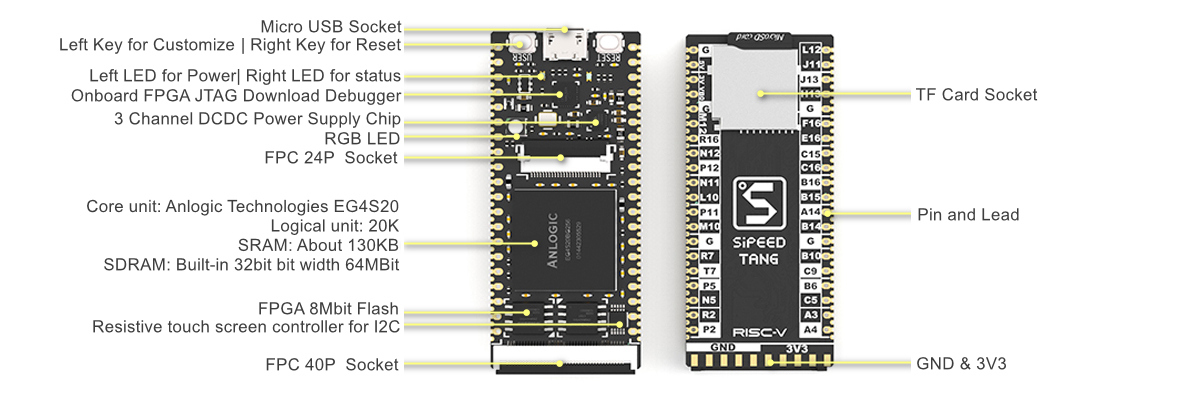The Lichee Tang Primer is a low-cost FPGA board made by Sipeed, using Anlogic’s EG4S20BG256 FPGA. It’s a great value for money, with 20,000 LUT4 logic elements and an onboard JTAG interface for uploading your bitstreams directly to the FPGA or SPI flash. In fact, it’s vendor IDE is especially user-friendly, being able to synthesise bitstreams in a matter of seconds, instead of minutes like Quartus/Vivado from the major players.

So, what are the downsides? Firstly, it’s just not well supported by the community or industry - meaning you’ll need to learn those pesky Quartus/Vivado tools eventually if you go into industry. Secondly, you’ll need to dig through documentation to use specific features (SERDES, ADC, PLL…) and vendor-provided IP.

However, for a beginner like me, we can wait to sort out those problems later… I just want to have a cheap and user-friendly platform to learn Verilog!

### Toolchain Setup

To set up the toolchain for this board, you can follow the official tutorial at the Sipeed wiki. I’ll briefly go through the setup steps:

3. For Linux, follow the setup guidelines here and run the `td -gui` command to open the IDE
4. For Windows, install using the executable and set your system time to be before 2018, to enable the provided Sipeed license. Then, you will be able to run the TD IDE with the ability to synthesise the bitstream
5. Install the USB drivers here
6. Double-check that your setup is valid by running the Blinky example

Edit: I’ve found that Anlogic has released a new, improved version of their IDE, feel free to try it out!

### Tutorial 1: FPGA Basics

As this series of tutorials were inspired by Nandland, I highly recommend you check out his videos before moving to the next few ones that actually involve implementing your designs on a physical FPGA.

For this tutorial, we will follow along with the first lecture of Nandland. Here, we will be setting up our development environment and writing a simple Verilog module and testbench with `iverilog` and `gtkwave`.

Firstly, we’ll want to install `iverilog` and `gtkwave`. `iverilog` is the Verilog compiler to perform simulations, and `gtkwave`allows you to view the resulting waveform.

#### Installing `iverilog` and `gtkwave`

For Windows, download the setup executable here. Run the installer and check the “Add to PATH” option to automatically add it to PATH, allowing you to call it from the terminal. This executable also allows you to install `gtkwave` at the same time.

For Linux, you can install from premade packages here. Follow the instructions for your distro. For Ubuntu, add the Universe repository to your `/etc/apt/sources.list` and run the command `sudo apt-get install iverilog gtkwave`.

#### Setting up Visual Studio Code

I personally prefer using Visual Studio Code (VSC) as my text editor for this series, as it has some community extensions that provide linting of Verilog code. Follow this guide to install VSC, and install the extension `mshr-h.veriloghdl`.

After installing the extension, go to `File >> Preferences >> Settings` and search for Verilog.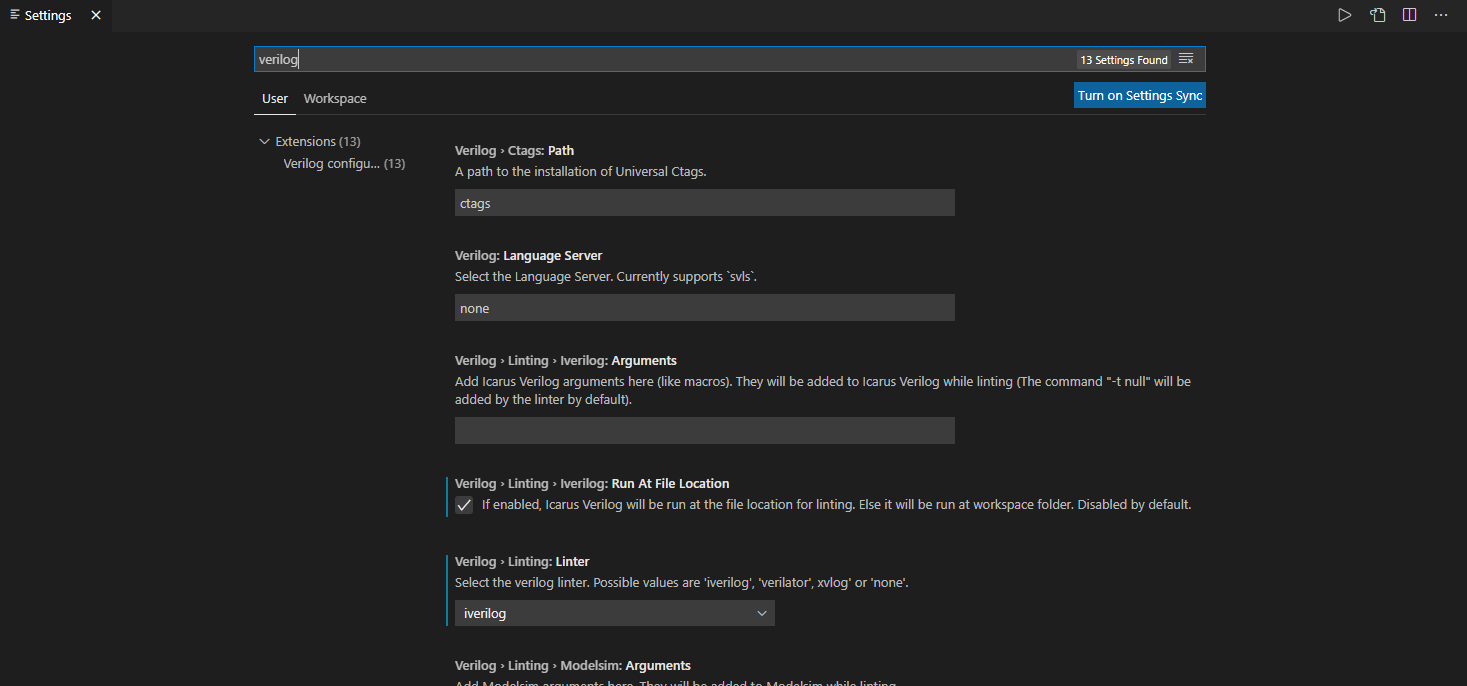Look for `Verilog >> Linting >> iverilog` and check that box. Then, select `iverilog` as your linter of choice. This will run `iverilog` at your code location and dynamically provide code completion and check for syntax errors. However, this does not check for logical errors, which you will need to debug using simulation.

#### Module Structure

In Verilog, a module is defined with the keyword `module`. The following is an example of how a module is defined.

``````module SwitchesToLEDs
(input i_Switch_1,
input i_Switch_2,
input i_Switch_3,
input i_Switch_4,
output o_LED_1,
output o_LED_2,
output o_LED_3,
output o_LED_4);

assign o_LED_1 = i_Switch_1;
assign o_LED_2 = i_Switch_2;
assign o_LED_3 = i_Switch_3;
assign o_LED_4 = i_Switch_4;

endmodule
``````

A module always starts with the keyword `module` followed by the name of the module. Following that, the `input` and `output` wires/registers to the module are defined. It’s good practice to label your variables appropriately, such as `i` for inputs and `o` for outputs. Remember to put the keyword `endmodule` at the end of your file.

Variables can have two main types in synthesizable Verilog: `wire` or `reg` for outputs and `wire` only for inputs. `wire` describes a physical connection between two ports, where any change in the driven state is propagated to the other side of the connection and is only compatible with combinational logic. `reg` is for driver states, where you can change the value of the variable using sequential logic as well.

The keyword `assign` can only be used with `wire` type variables, thereby driving the signal continuously. These will always be active, not just at the clock edge.

This example from Nandland illustrates how you can take several button inputs and directly connect them to LED outputs.

#### Logic Gates

Now that we’ve taken a look at this basic example, let’s try to modify it with some logic gates.

``````// Logic gate examples
module SwitchesToLEDs
(input i_Switch_1,
input i_Switch_2,
output o_LED_1,
output o_LED_2,
output o_LED_3,
output o_LED_4);

assign o_LED_1 = i_Switch_1 & i_Switch_2;     // AND  gate
assign o_LED_2 = i_Switch_1 | i_Switch_2;     // OR   gate
assign o_LED_3 = ~(i_Switch_1 & i_Switch_2);  // NAND gate
assign o_LED_4 = i_Switch_1 ^  i_Switch_2;    // XOR  gate

endmodule
``````

In this example, we use the bitwise operators `&`, `|`, `~` and `^` for AND, OR, NOT and XOR. It is also possible to use full logical operators such as `&&`, `||` and `!` for AND, OR and NOT. A deep dive into operators is available here.

#### Creating a Testbench for Simulation

Now, let’s save our file as `SwitchesToLEDs.v`. Create a new file for our testbench called `SwitchesToLEDs_tb.v`. At the start of the file, we will define the timescale for which the simulation is done over, which is the duration of one clock pulse.

```````timescale 1ns/1ns
``````

Following that, we will need to include the source Verilog file of our module.

```````include "SwitchesToLEDs.v"
``````

Then, we will create our testbench module. Testbenches don’t contain inputs or outputs, hence there are no brackets containing them.

```````timescale 1ns/1ns
`include "SwitchesToLEDs.v"

module SwitchesToLEDs_tb;
// Code for testbench here
endmodule
``````

Now we create the inputs and outputs for our module, which we call the Unit Under Test (UUT). `reg` for inputs and `wire` for outputs, the reverse of what we declared in the actual module. This allows us to drive the inputs and read the outputs of the UUT.

```````timescale 1ns/1ns
`include "SwitchesToLEDs.v"

module SwitchesToLEDs_tb;
reg i_Switch_1;
reg i_Switch_2;
wire o_LED_1;
wire o_LED_2;
wire o_LED_3;
wire o_LED_4;

// Code for testbench here
endmodule
``````

Next, we instantiate the UUT. When instantiating a module, the format is `moduleName (parameters) InstanceName (inputs/outputs)`. You can provide the inputs/outputs in order, or you can use their internal variable names to match them, as shown below.

```````timescale 1ns/1ns
`include "SwitchesToLEDs.v"

module SwitchesToLEDs_tb;
reg i_Switch_1;
reg i_Switch_2;
wire o_LED_1;
wire o_LED_2;
wire o_LED_3;
wire o_LED_4;

// Instantiating module to test
SwitchesToLEDs uut(
.i_Switch_1(i_Switch_1),
.i_Switch_2(i_Switch_2),
.o_LED_1(o_LED_1),
.o_LED_2(o_LED_2),
.o_LED_3(o_LED_3),
.o_LED_4(o_LED_4)
);
// Code for testbench here
endmodule
``````

Now, let’s initialise the testbench procedure that we want to conduct. The `initial` keyword allows us to define behaviour that only happens once, at the beginning. Verilog doesn’t use curly braces to detect code blocks, rather it uses `begin` and `end` keywords.

To output the simulated values of all testbench variables, use the command `\$dumpfile()` and `\$dumpvars()` to save them into a `vcd` format readable by `gtkwave`.

```````timescale 1ns/1ns
`include "SwitchesToLEDs.v"

module SwitchesToLEDs_tb;
reg i_Switch_1;
reg i_Switch_2;
wire o_LED_1;
wire o_LED_2;
wire o_LED_3;
wire o_LED_4;

// Instantiating module to test
SwitchesToLEDs uut(
.i_Switch_1(i_Switch_1),
.i_Switch_2(i_Switch_2),
.o_LED_1(o_LED_1),
.o_LED_2(o_LED_2),
.o_LED_3(o_LED_3),
.o_LED_4(o_LED_4)
);

initial begin
// Define testbench behaviour
\$dumpfile("SwitchesToLEDs_tb.vcd");
\$dumpvars(0, SwitchesToLEDs_tb);

// Code for testbench here
end

endmodule
``````

Since we want to test our AND, OR, NAND and XOR gate behavior, let’s create a truth table for the expected outputs for every given set of inputs. For simplicity, let’s call the switches A and B respectively, and outputs C, D, E, F.

A B C D E F
0 0 0 0 1 0
1 0 0 1 1 1
0 1 0 1 1 1
1 1 1 1 0 0

Setting these inputs respectively, with a delay of 10 timesteps using `#10` in the testbench. The curly bracket notation groups signals into buses from left to right, Most Significant Bit to Least Significant Bit.

```````timescale 1ns/1ns
`include "SwitchesToLEDs.v"

module SwitchesToLEDs_tb;
reg i_Switch_1;
reg i_Switch_2;
wire o_LED_1;
wire o_LED_2;
wire o_LED_3;
wire o_LED_4;

// Instantiating module to test
SwitchesToLEDs uut(
.i_Switch_1(i_Switch_1),
.i_Switch_2(i_Switch_2),
.o_LED_1(o_LED_1),
.o_LED_2(o_LED_2),
.o_LED_3(o_LED_3),
.o_LED_4(o_LED_4)
);

initial begin
// Define testbench behaviour
\$dumpfile("SwitchesToLEDs_tb.vcd");
\$dumpvars(0, SwitchesToLEDs_tb);

// Test conditions
{i_Switch_1, i_Switch_2} = 2'b00; #10;
{i_Switch_1, i_Switch_2} = 2'b10; #10;
{i_Switch_1, i_Switch_2} = 2'b01; #10;
{i_Switch_1, i_Switch_2} = 2'b11; #10;
end

endmodule
``````

Knowing that our test cases are sequentially incrementing, we can use a `for` loop using an `integer` variable. However, keep in mind that these constructs are not synthesizable and cannot be used in your main module. Keep in mind that `++` is not valid to increment your counter. Use `\$display()` to print messages to terminal.

```````timescale 1ns/1ns
`include "SwitchesToLEDs.v"

module SwitchesToLEDs_tb;
reg i_Switch_1;
reg i_Switch_2;
wire o_LED_1;
wire o_LED_2;
wire o_LED_3;
wire o_LED_4;

// Instantiating module to test
SwitchesToLEDs uut(
.i_Switch_1(i_Switch_1),
.i_Switch_2(i_Switch_2),
.o_LED_1(o_LED_1),
.o_LED_2(o_LED_2),
.o_LED_3(o_LED_3),
.o_LED_4(o_LED_4)
);

initial begin
// Define testbench behaviour
\$dumpfile("SwitchesToLEDs_tb.vcd");
\$dumpvars(0, SwitchesToLEDs_tb);

// Test conditions
for (integer i=0; i<4; i = i+1) begin
{i_Switch_1, i_Switch_2} = i;
#10;
end

\$display("Test completed!");
end

endmodule
``````

With this, we have finished our Verilog testbench. To run it and generate the `vvp` file, use the following command:

``````iverilog -o SwitchesToLEDs_tb.vvp SwitchesToLEDs_tb.v
``````

Then, create the output `vcd` file.

``````vvp SwitchesToLEDs_tb.vvp
``````

Now, let’s open `gtkwave` by typing that in a terminal, bringing up the GUI. Click on `File >> Open New Tab` and find your `.vcd` output file.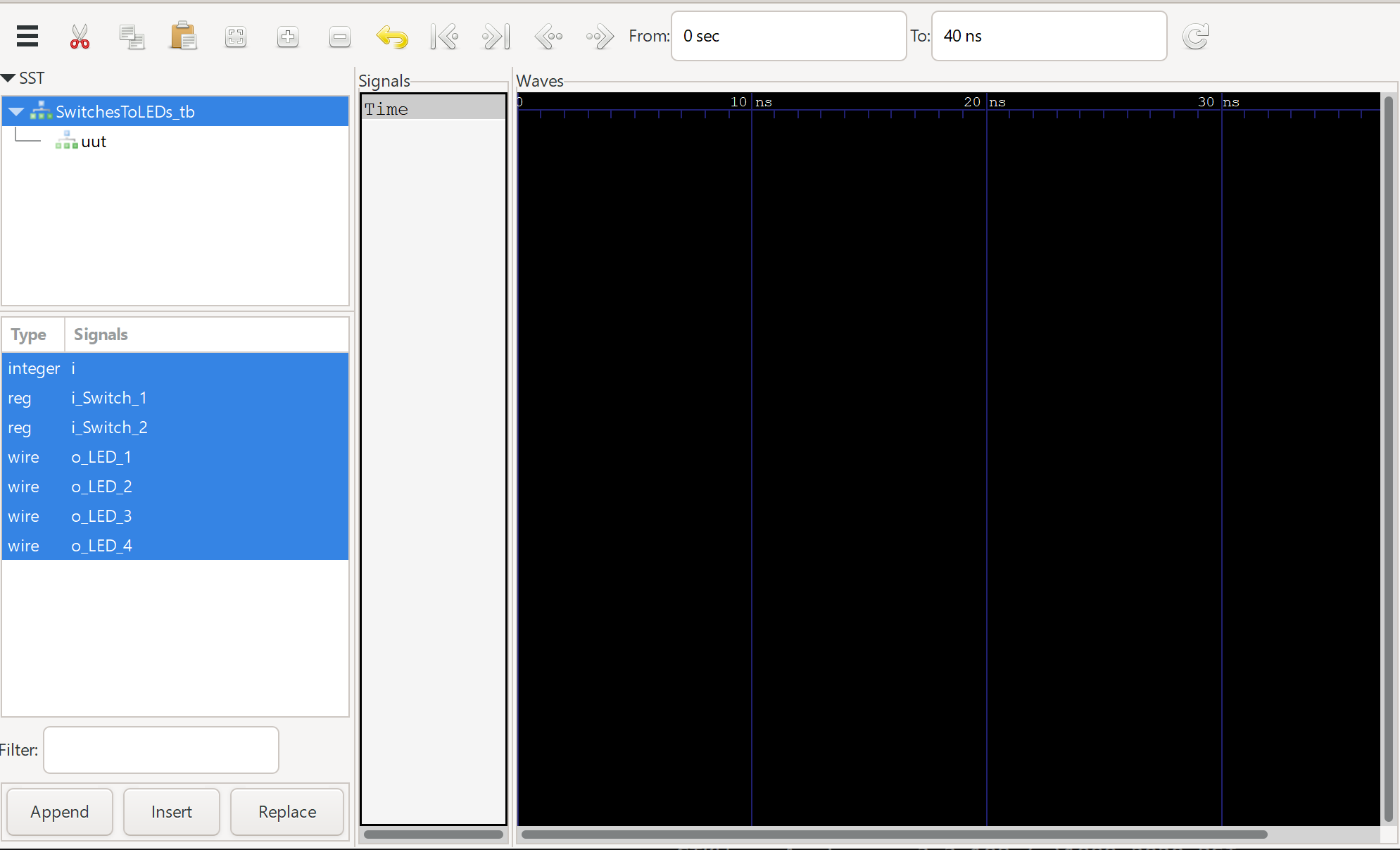Select all your signals by clicking on the top one, then Shift+click on the bottom to select all. Click `Append` to add them to the waveform viewer.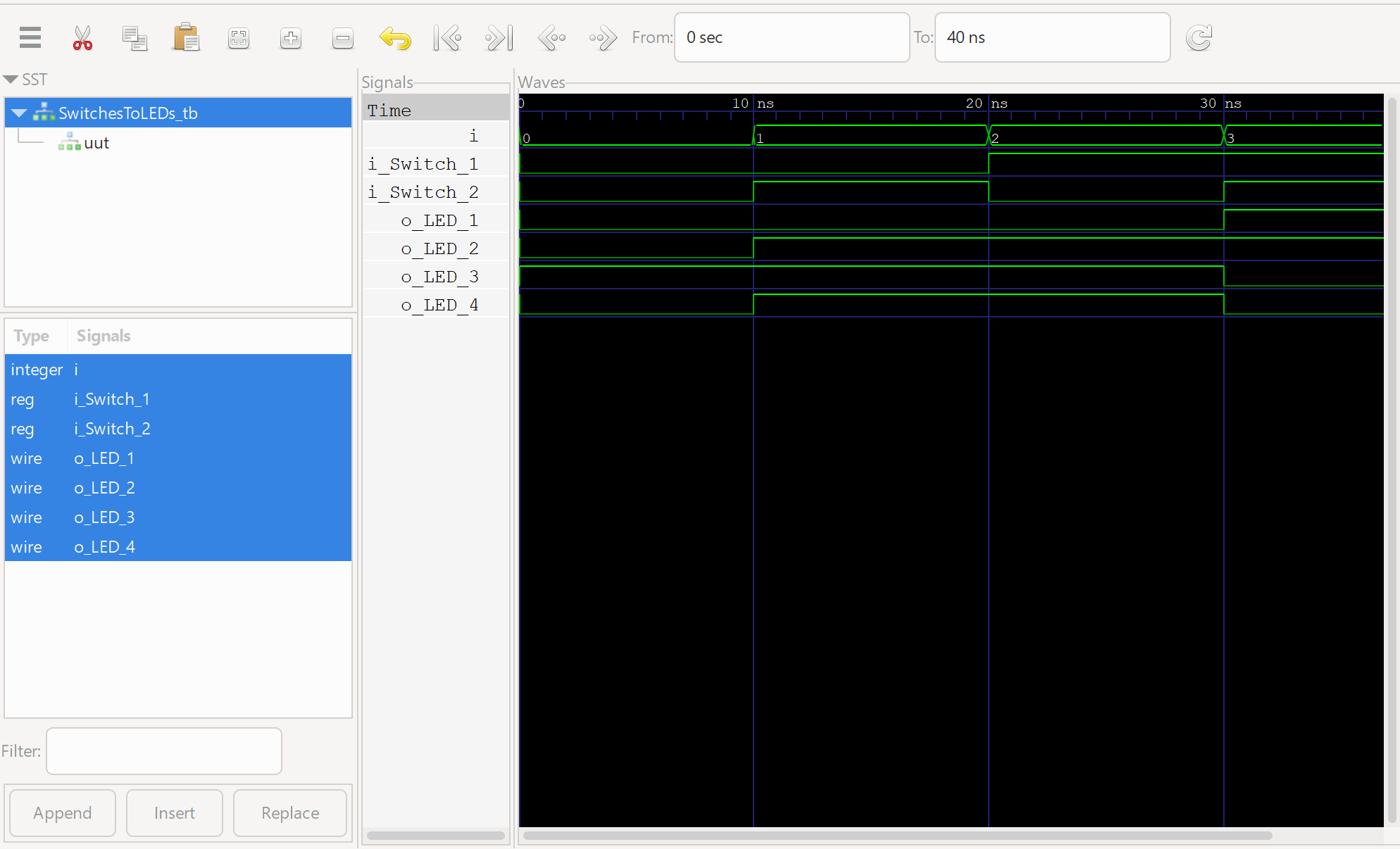Comparing the result of our waveforms to the truth table, we see that everything is working fine! Look horizontal across the truth table and vertical down the waveform for a 1:1 comparison in this case.

#### Deploying to hardware

Now that we know our program works fine with the simulator, let’s deploy it on actual hardware! Open up the Tang Dynasty IDE using either `td -gui` in Linux or through your TimeAsDate program in Windows.

Right-click the Project menu and click on `New Project`.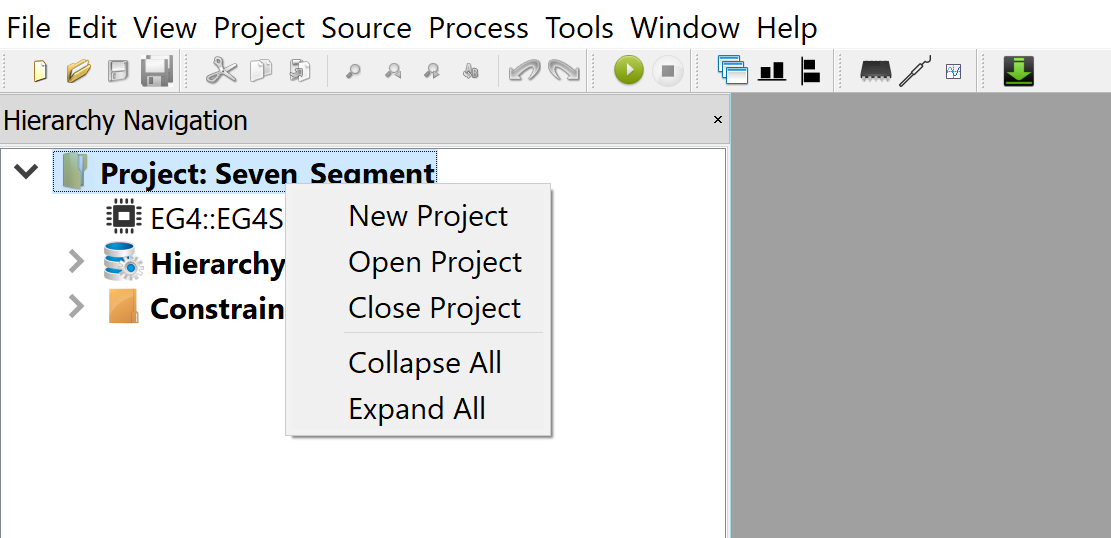Select the correct device name for the board, `EG4X20BG256`.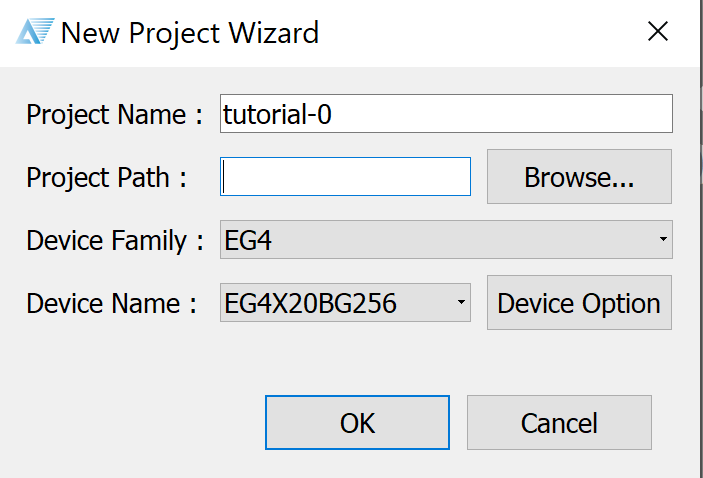If you’ve saved your Verilog source file in the same directory, you can click `Add Sources` to add your source file to the project. If not, click `New Source` and paste in your Verilog code from `SwitchesToLEDs.v`.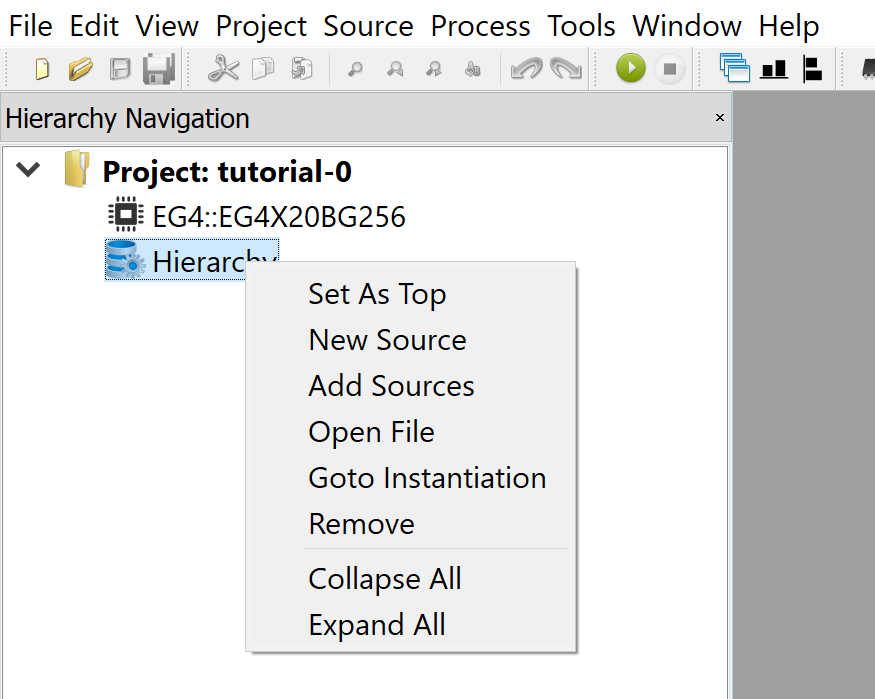The IDE will automatically set your only source file as the Top module. In a Verilog design, the project starts from the Top module, which contains instantiations of all other modules in the hierarchy. We’ll touch on this in a later tutorial where we combine multiple Verilog source files into a single design.

Now, we’ve got to define our Constraints file, which defines how these pins are connected to external I/O on the FPGA. In your text editor, create a file called `io.adc` and save it with the following contents. `LOCATION` defines the external pin, `IOSTANDARD` defines the voltage logic levels and `DRIVESTRENGTH` defines the current driver strength in mA.

``````set_pin_assignment	{ i_Switch_1 }	{ LOCATION = A4; IOSTANDARD = LVCMOS33; }
set_pin_assignment	{ i_Switch_2 }	{ LOCATION = A3; IOSTANDARD = LVCMOS33; }
set_pin_assignment	{ o_LED_1 }	{ LOCATION = C5; IOSTANDARD = LVCMOS33; DRIVESTRENGTH = 20; }
set_pin_assignment	{ o_LED_2 }	{ LOCATION = B6; IOSTANDARD = LVCMOS33; DRIVESTRENGTH = 20; }
set_pin_assignment	{ o_LED_3 }	{ LOCATION = C9; IOSTANDARD = LVCMOS33; DRIVESTRENGTH = 20; }
set_pin_assignment	{ o_LED_4 }	{ LOCATION = B10; IOSTANDARD = LVCMOS33; DRIVESTRENGTH = 20; }
``````

If you are making your own connections, refer to the schematic for the correct `LOCATION`.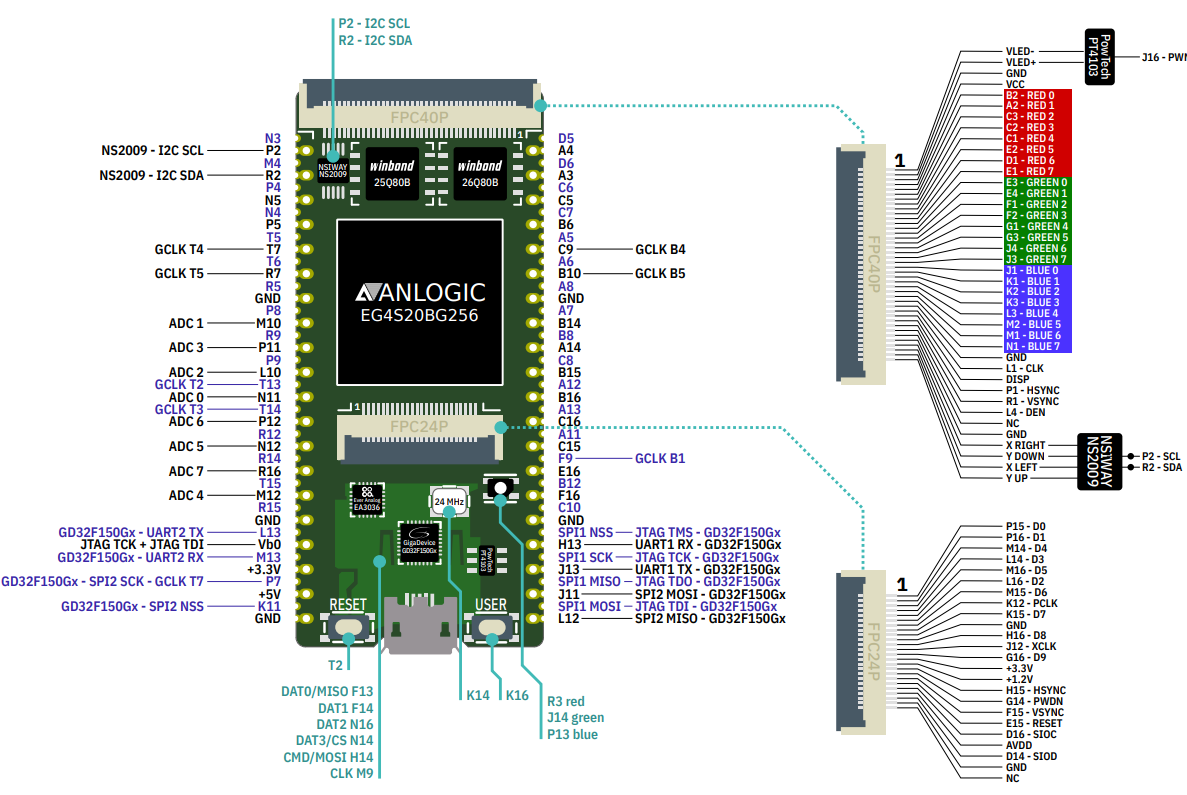Right-click `Constraints` and `Add ADC File`.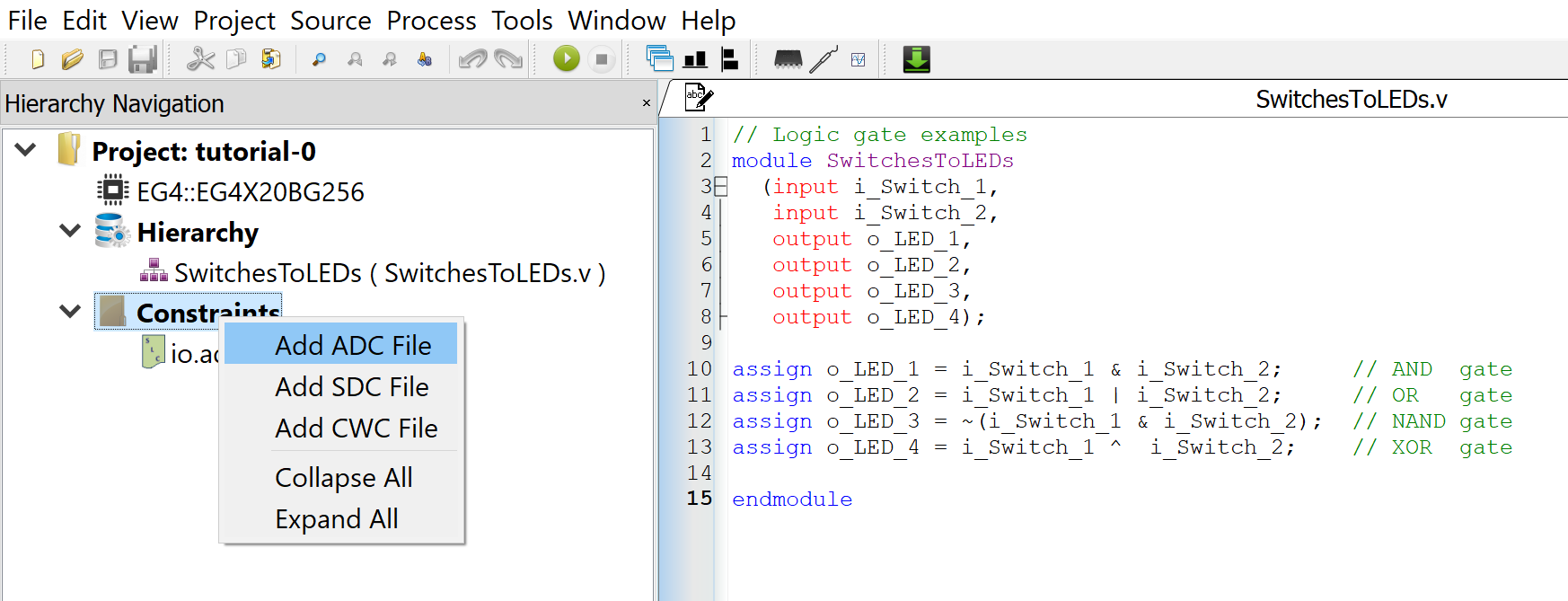Now, double click on `Generate Bitstream` to start the whole process of synthesis, place-and-route, and implementation. Alternatively, you can step through this process one at a time.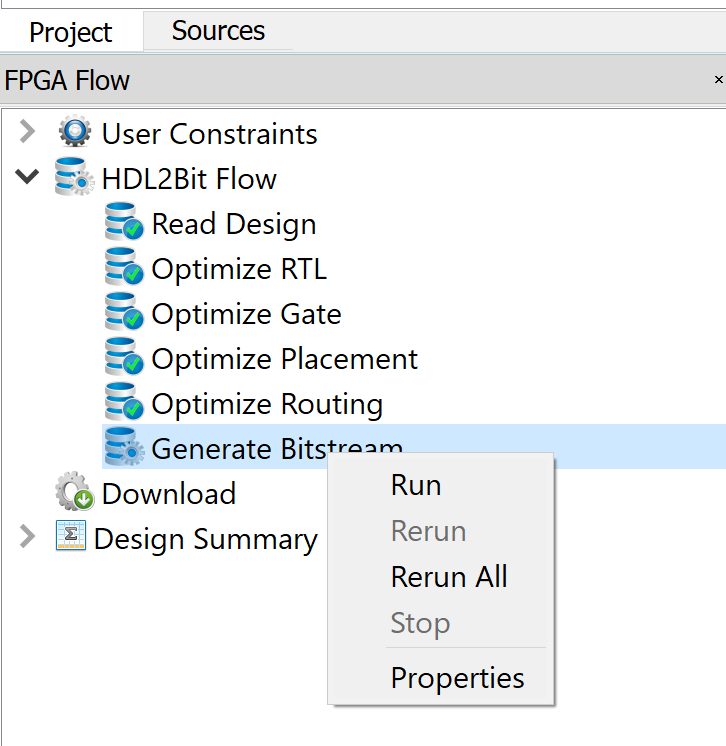This will generate a `.bit` file, the bitstream to be uploaded to the board. Double-click on `Download` and `Add` the file. Click `Run` to upload the file directly to the FPGA. As the FPGA fabric is volatile, it will lose its configuration when powered off. To keep it, you will need to `Create Flash File` and upload that file to the flash instead. We won’t be covering that here as it’s not immediately useful for learning purposes. You should see the same behaviour observed in your simulation.

Congratulations! You’ve done your first FPGA project and you’re well on your way down a rabbit hole of programmable logic fun!

### Tutorial 2: Seven Segment Display

In this tutorial, we will control a seven segment display using the FPGA. This will introduce concepts such as `module` instantiation where code can be written and reused, a similar paradigm to Object Oriented Programming. Click here for the tutorial.

### Tutorial 3: UART Interface

In this tutorial, we will create a UART interface to send and receive data with your computer. This introduces a state machine to handle incoming data, and how to break down complex logic into states for easier management. Click here for the tutorial.

### Tutorial 4: FIFO Buffer

This introduces the concept of a First In First Out (FIFO) buffer between the external UART interface and the internal FPGA logic. This is necessary as the UART peripheral and internal FPGA logic work in different clock domains, and may not always be available to receive data when it is transmitted/received. Click here for the tutorial.

### Tutorial 5: VGA Interface

In this tutorial, we will explore the VGA specification to send RGB video data out to a monitor. This should work with any old or modern monitor. Modern monitors may rescale your image to fit the 16:9 aspect ratio. Click here for the tutorial.

### Tutorial 6: HDMI Interface

This expands on the previous tutorial to send RGB video data out to a monitor through HDMI. DVI/HDMI demands much higher clock speeds, and this is where we introduce the concept of a Phase Locked Loop (PLL), to generate faster clock signals. Click here for the tutorial.

### References

For this tutorial I referenced the following sources:

# Algothon 2021 - ML in Finance## Algothon 2021 - ML in FinanceI participated in Algothon 2021, organised by the Imperial College Algorithmic Trading Society and Aspect Capital. This competition had us creating prediction models, low-latency algorithms, and even a dashboard for financial modelling. It was also my first solo hackathon (as I was based in a different timezone from most participants), which kept the pressure up as I attempted to solve most challenges. My codebase is available here.

### Data Cleaning

For this challenge, I went with the simple approach of removing outliers with an adaptive threshold. However, that didn’t turn out well as the grading criteria was using the Mean Squared Error (MSE) of the cleaned vs original “clean” dataset. This meant that removing data points was not an option; I should have normalised detected outliers using a rolling median.

### Prediction

This challenge involved predicting the Log-Returns value of provided stocks over a time period of a few years. In essence - picking the correct stocks to buy in the market, and when to sell them - what actual quantitative analysts do at work.

I used Keras’ sequential class to build up a regression model, using all 177 features as the input. However, I found that accuracy was poor in this configuration due to noise from excessive input features. Using Scikit-learn’s Feature Selector to cut down the number of input features would be a first step in improving the accuracy of the model, by removing less correlated features.

### Data Visualisation

The data visualisation task involved showcasing the dataset from the Data Cleaning Challenge. A simple graph was built using Dash Plotly in python, with a simple interactive slider to calculate market momentum using a simple moving average. The demo is available here.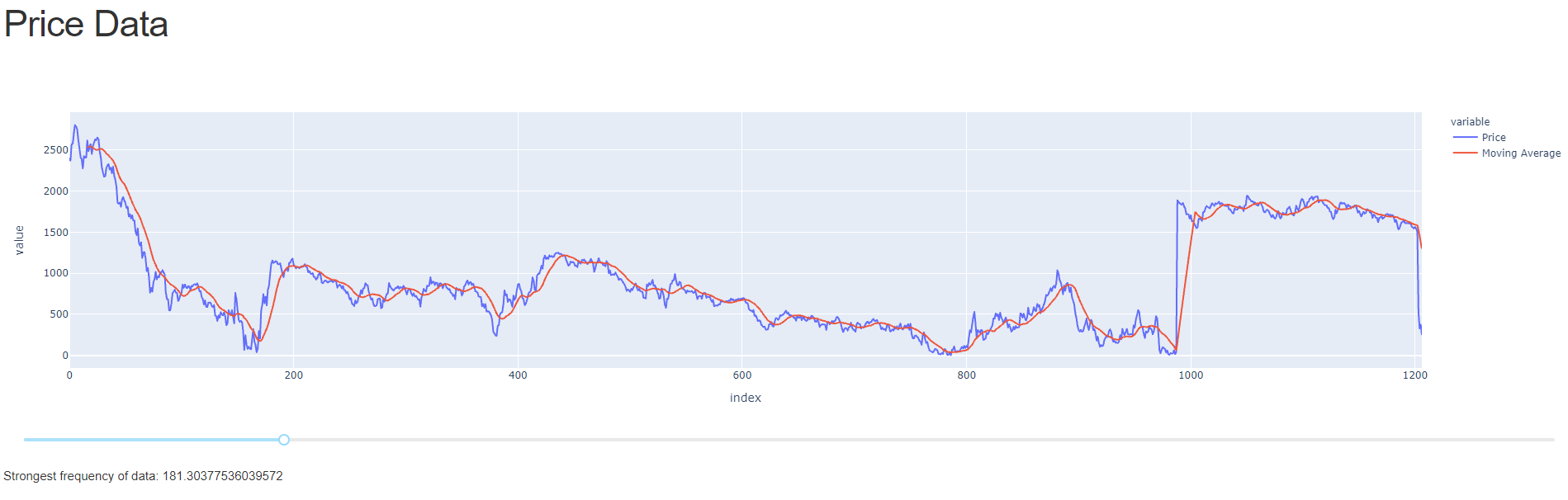### Low Latency Challenge

This challenge involves predicting the rise/fall of a stock price in the next tick. Due to the high-frequency nature of the prediction and underlying data, an accuracy of >50% was requested. Latency was the key here.

The provided training set was a 1826 long time-series data of Log-Returns, which was split into sets of 500 for training purposes.

The algorithm was tested with the dataset provided. After further analysis, it was recognised that the algorithm was not producing consistent results of >50% accuracy. To optimise for speed, a barebones script was used as a Constant Guesser.

The program was written in C++ to minimise latency, with an emphasis on runtime speed and minimal focus on accuracy. The code was tested on an RPI 4 4GB, with a compile time of 11s and a runtime of 9ms (mostly due to the `cat` write calls).

As an attempt to bring latency down to its minimum, I submitted the program `echo 1` to guess my way to victory with a runtime in the microseconds (not including `cat`) however that didn’t work out as my program failed to run on the organiser’s testbench for an unknown reason.

# Brainhack 2020 - NLP and CV in Robotics## DSTA Brainhack 2020: NLP/CV in Robotics

I engineered a cooperative robotics platform where a drone flies to a designated point to take a photo of the arena and plot a course around the obstacles for the ground robot using OpenCV. The robot would then use dead reckoning to navigate the course. In the second stage, we trained a Natural Language Processing (NLP) model to identify a doll based on its description and a Computer Vision (CV) model using YoloV3 in PyTorch to identify said doll in the arena. Once identified, the robot would pick up the doll with its grabber and score points for the competition. My team and I placed 4th overall.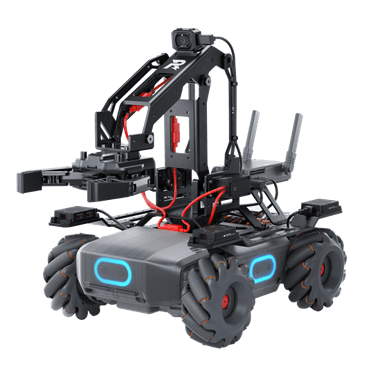Cooperative robotics was no issue for us, as we were easily able to characterise the shape of the track and start/end points using feature detection in OpenCV. The drone would fly above the track, grab a picture, send it back to the computer for processing, which would issue instructions to the robot via the vendor API over TCP.

The main difficulty that we encountered was the system integration between the NLP and CV parts of the competition. Our NLP was good enough to extract the correct description of the doll from the sentence, but was unable to successfully identify the doll in the arena. We had previously tested these two functions separately, but never as a whole. Unfortunately, this mistake ultimately cost us the trophy.